The Australian Journal of Mathematical Analysis and Applications

 Home News Editors Volumes RGMIA Subscriptions Authors Contact

ISSN 1449-5910

You searched for chen
Total of 374 results found in site

32: Paper Source PDF document

Paper's Title:

An Integration Technique for Evaluating Quadratic Harmonic Sums

Author(s):

J. M. Campbell and K.-W. Chen

Department of Mathematics and Statistics,
York University, 4700 Keele St, Toronto,
ON M3J 1P3,
E-mail: jmaxwellcampbell@gmail.com

Department of Mathematics, University of Taipei,
Taipei 10048, Taiwan.
E-mail: kwchen@uTaipei.edu.tw
URL: https://math.utaipei.edu.tw/p/412-1082-22.php

Abstract:

The modified Abel lemma on summation by parts has been applied in many ways recently to determine closed-form evaluations for infinite series involving generalized harmonic numbers with an upper parameter of two. We build upon such results using an integration technique that we apply to ``convert'' a given evaluation for such a series into an evaluation for a corresponding series involving squared harmonic numbers.

9: Paper Source PDF document

Paper's Title:

L∞- Error Estimate of Schwarz Algorithm for Elliptic Quasi-Variational Inequalities Related to Impulse Control Problem

Author(s):

Lab. LANOS, Department of Mathematics,
P.O.Box 12, Annaba 23000,
Algeria.

Lab. LAIG, Department of Mathematics,
University May 8th 1945,
P.O.Box 401, Guelma 24000,
Algeria.

allmehri@yahoo.fr

Abstract:

In this work, we study Schwarz method for a class of elliptic quasi-variational inequalities. The principal result of this investigation is to prove the error estimate in ∞-norm for two domains with overlapping nonmatching grids, using the geometrical convergence, and the uniform convergence of Cortey Dumont.

9: Paper Source PDF document

Paper's Title:

Ostrowski Type Inequalities for Lebesgue Integral: a Survey of Recent Results

Author(s):

Sever S. Dragomir1,2

1Mathematics, School of Engineering & Science
Victoria University, PO Box 14428
Melbourne City, MC 8001,
Australia
E-mail: sever.dragomir@vu.edu.au

2DST-NRF Centre of Excellence in the Mathematical and Statistical Sciences,
School of Computer Science & Applied Mathematics,
University of the Witwatersrand,
Private Bag 3, Johannesburg 2050,
South Africa
URL: http://rgmia.org/dragomir

Abstract:

The main aim of this survey is to present recent results concerning Ostrowski type inequalities for the Lebesgue integral of various classes of complex and real-valued functions. The survey is intended for use by both researchers in various fields of Classical and Modern Analysis and Mathematical Inequalities and their Applications, domains which have grown exponentially in the last decade, as well as by postgraduate students and scientists applying inequalities in their specific areas.

8: Paper Source PDF document

Paper's Title:

Inequalities Relating to the Gamma Function

Author(s):

Chao-Ping Chen and Feng Qi

Department of Applied Mathematics and Informatics, Research Institute of Applied Mathematics,
Henan Polytechnic University, Jiaozuo City, Henan 454000, China

chenchaoping@hpu.edu.cn

Department of Applied Mathematics and Informatics, Research Institute of Applied Mathematics,
Henan Polytechnic University, Jiaozuo City, Henan 454000, China

qifeng@hpu.edu.cn, fengqi618@member.ams.org

U
rl: http://rgmia.vu.edu.au/qi.html, http://dami.hpu.edu.cn/qifeng.html

Abstract:

For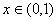, we have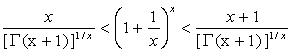.

For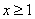,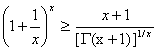,

And equality occurs for x=1.

8: Paper Source PDF document

Paper's Title:

On an Extension of Hilbert’s Integral Inequality with Some Parameters

Author(s):

Bicheng Yang

Department of Mathematics, Guangdong Education College, Guangzhou, Guangdong 510303, People’s Republic of China.
URL
:

Abstract:

In this paper, by introducing some parameters and estimating the weight function, we give an extension of Hilbert’s integral inequality with a best constant factor. As applications, we consider the equivalent form and some particular results.

7: Paper Source PDF document

Paper's Title:

On Vector Variational Inequality Problem in Terms of Bifunctions

Author(s):

C. S. Lalitha and Monika Mehta

Department of Mathematics, Rajdhani College,
University of Delhi, Raja Garden,
Delhi 110015, India
cslalitha@rediffmail.com

Department of Mathematics, Satyawati College,
University Of Delhi, Ashok Vihar,
Phase-III, Delhi 110052, India
mridul_in@yahoo.com

Abstract:

In this paper, we consider a generalized vector variational inequality problem expressed in terms of a bifunction and establish existence theorems for this problem by using the concepts of cone convexity and cone strong quasiconvexity and employing the celebrated Fan's Lemma. We also give two types of gap functions for this problem.

7: Paper Source PDF document

Paper's Title:

A Wallis Type Inequality and a Double Inequality for Probability Integral

Author(s):

Jian Cao, Da-Wei Niu and Feng Qi

School of Mathematics and Informatics,
Henan Polytechnic University, Jiaozuo City,
Henan Province, 454010,
China
21caojian@163.com

School of Mathematics and Informatics,
Henan Polytechnic University, Jiaozuo City,
Henan Province, 454010,
China
nnddww@tom.com

Research Institute of Mathematical Inequality Theory,
Henan Polytechnic University, Jiaozuo City,
Henan Province, 454010,
China
qifeng@hpu.edu.cn
fengqi618@member.ams.org
qifeng618@hotmail.com
qifeng618@msn.com
qifeng618@qq.com
URL: http://rgmia.vu.edu.au/qi.html

Abstract:

In this short note, a Wallis type inequality with the best upper and lower bounds is established. As an application, a double inequality for the probability integral is found.

7: Paper Source PDF document

Paper's Title:

Iterative Algorithm for Split Generalized Mixed Equilibrium Problem Involving Relaxed Monotone Mappings in Real Hilbert Spaces

Author(s):

1U.A. Osisiogu, F.L. Adum, and 2C. Izuchukwu

1Department of Mathematics and Computer Science,
Ebonyi State University, Abakaliki,
Nigeria.

2School of Mathematics, Statistics and Computer Science,
University of KwaZulu-Natal, Durban,
South Africa.
E-mail: izuchukwuc@ukzn.ac.za, izuchukwu_c@yahoo.com

Abstract:

The main purpose of this paper is to introduce a certain class of split generalized mixed equilibrium problem involving relaxed monotone mappings. To solve our proposed problem, we introduce an iterative algorithm and obtain its strong convergence to a solution of the split generalized mixed equilibrium problems in Hilbert spaces. As special cases of the proposed problem, we studied the proximal split feasibility problem and variational inclusion problem.

7: Paper Source PDF document

Paper's Title:

Differential Equations for Indicatrices, Spacelike and Timelike Curves

Author(s):

Department of Mathematics,
Jaypee University of Information Technology,
India.
E-mail: sksameer08@gmail.com, pandeypkdelhi@gmail.com

Abstract:

Motivated by the recent work of Deshmukh et al. , in this paper we show that Tangent, Binormal, and Principal Normal indicatrices do not form non-trivial differential equations. Finally, we obtain the 4th-order differential equations for spacelike and timelike curves.

6: Paper Source PDF document

Paper's Title:

On a Hilbert-type Inequality with the Polygamma Function

Author(s):

Bing He and Bicheng Yang
Department of Mathematics, Guangdong Education College,
Guangzhou, 510303,
China.

Department of Mathematics, Guangdong Education College,
Guangzhou, 510303,
China.

Abstract:

By applying the method of weight function and the technique of real analysis, a Hilbert-type inequality with a best constant factor is established, where the best constant factor is made of the polygamma function. Furthermore, the inverse form is given.

6: Paper Source PDF document

Paper's Title:

Stability of an Almost Surjective epsilon-Isometry in The Dual of Real Banach Spaces

Author(s):

Minanur Rohman, Ratno Bagus Edy Wibowo, Marjono

Department of Mathematics, Faculty of Mathematics and Natural Sciences,
Brawijaya University,
Jl. Veteran Malang 65145,
Indonesia.
E-mail: miminanira@gmail.com

Department of Mathematics, Faculty of Mathematics and Natural Sciences,
Brawijaya University,
Jl. Veteran Malang 65145,
Indonesia.
E-mail: rbagus@ub.ac.id

Department of Mathematics, Faculty of Mathematics and Natural Sciences,
Brawijaya University,
Jl. Veteran Malang 65145,
Indonesia.
E-mail: marjono@ub.ac.id

Abstract:

In this paper, we study the stability of epsilon-isometry in the dual of real Banach spaces. We prove that the almost surjective epsilon-isometry mapping is stable in dual of each spaces. The proof uses Gâteaux differentiability space (GDS), weak-star exposed points, norm-attaining operator, and some studies about epsilon-isometry that have been done before.

6: Paper Source PDF document

Paper's Title:

Conservativeness Criteria of Girsanov Transformation for Non-Symmetric Jump-diffusion

Author(s):

Mila Kurniawaty

DDepartment of Mathematics,
Universitas Brawijaya, Malang,
Indonesia.
E-mail: mila_n12@ub.ac.id

Abstract:

We develop the condition in our previous paper [The Conservativeness of Girsanov transformed for symmetric jump-diffusion process (2018)] in the framework of nonsymmetric Markov process with jumps associated with regular Dirichlet form. We prove the conservativeness of it by relation in duality of Girsanov transformed process and recurrent criteria of Dirichlet form.

5: Paper Source PDF document

Paper's Title:

Logarithmically complete monotonicity properties for the gamma functions

Author(s):

Chao-Ping Chen and Feng Qi

Department of Applied Mathematics and Informatics,
Research Institute of Applied Mathematics,
Henan Polytechnic University,
Jiaozuo City, Henan 454010,
China.
chenchaoping@hpu.edu.cn

Department of Applied Mathematics and Informatics,
Research Institute of Applied Mathematics,
Henan Polytechnic University,
Jiaozuo City, Henan 454010,
China.
qifeng@hpu.edu.cn
fengqi618@member.ams.org

Abstract:

Some logarithmically completely monotonic functions involving the gamma functions are presented. As a consequence, some known results are proved and refined.

5: Paper Source PDF document

Paper's Title:

Oscillations of First Order Linear Delay Difference Equations

Author(s):

G. E. Chatzarakis and I. P. Stavroulakis

Department of Mathematics, University of Ioannina,
451 10, Greece
ipstav@cc.uoi.gr

Abstract:

Consider the first order linear delay difference equation of the form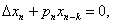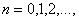where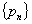is a sequence of nonnegative real numbers, k is a positive integer and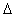denotes the forward difference operator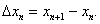New oscillation criteria are established when the well-known oscillation conditions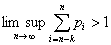and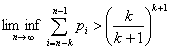are not satisfied. The results obtained essentially improve known results in the literature.

5: Paper Source PDF document

Paper's Title:

A note on Inequalities due to Martins, Bennett and Alzer

Author(s):

József Sándor

Babeş-Bolyai University of Cluj, Department of Mathematics and Computer Sciences
Kogălniceanu Nr.1, Cluj-Napoca,
Romania.
jjsandor@hotmail.com
jsandor@member.ams.org

Abstract:

A short history of certain inequalities by Martins, Bennett as well as Alzer, is provided. It is shown that, the inequality of Alzer for negative powers , or Martin's reverse inequality  are due in fact to Alzer . Some related results, as well as a conjecture, are stated.

5: Paper Source PDF document

Paper's Title:

Uniform Convergence of Schwarz Method for Noncoercive Variational Inequalities Simple Proof

Author(s):

Department of mathematics, LANOS Laboratory,
Faculty of the Sciences, University Badji Mokhtar,
P.O 23000 Annaba,
Algeria.

haiourm@yahoo.fr,

Abstract:

In this paper we study noncoercive variational inequalities, using the Schwarz method. The main idea of this method consists in decomposing the domain in two subdomains. We give a simple proof for the main result concerning L error estimates, using the Zhou geometrical convergence and the L approximation given for finite element methods by Courty-Dumont.

5: Paper Source PDF document

Paper's Title:

Common Fixed Point Results for Banach Operator Pairs and Applications to Best Approximation

Author(s):

Hemant Kumar Nashine

Department of Mathematics,
Disha Institute of Management and Technology,
Satya Vihar, Vidhansabha - Chandrakhuri Marg (Baloda Bazar Road), Mandir Hasaud,
Raipur - 492101(Chhattisgarh), India.

hemantnashine@rediffmail.com
nashine_09@rediffmail.com

Abstract:

The common fixed point results for Banach operator pair with generalized nonexpansive mappings in q-normed space have been obtained in the present work. As application, some more general best approximation results have also been determined without the assumption of linearity or affinity of mappings. These results unify and generalize various existing known results with the aid of more general class of noncommuting mappings.

5: Paper Source PDF document

Paper's Title:

Coefficient Estimates Of Sakaguchi Kind Functions Using Lucas Polynomials

Author(s):

H. Priya and B. Srutha Keerthi

Department of Mathematics,
VIT Chennai Campus,
Chennai - 600 048,
India.
E-mail: priyaharikrishnan18@gmail.com

Department of Mathematics,
VIT Chennai Campus,
Chennai - 600 048,
India.
E-mail: i
sruthilaya06@yahoo.co.in

Abstract:

By means of (p,q) Lucas polynomials, we estimate coefficient bounds and Fekete-Szego inequalities for functions belonging to this class. Several corollaries and consequences of the main results are also obtained.

5: Paper Source PDF document

Paper's Title:

Oscillatory Behavior of Second-Order Non-Canonical Retarded Difference Equations

Author(s):

G.E. Chatzarakis1, N. Indrajith2, E. Thandapani3 and K.S. Vidhyaa4

1Department of Electrical and Electronic Engineering Educators,
School of Pedagogical and Technological Education,
Marousi 15122, Athens,
Greece.
E-mail:  gea.xatz@aspete.gr, geaxatz@otenet.gr

2Department of Mathematics,
Presidency College, Chennai - 600 005,
India.
E-mail: indrajithna@gmail.com

3Ramanujan Institute for Advanced Study in Mathematics,
Chennai - 600 005,
India.
E-mail: ethandapani@yahoo.co.in

4Department of Mathematics,
SRM Easwari Engineering College,
Chennai-600089,
India.
E-mail: vidyacertain@gmail.com

Abstract:

Using monotonic properties of nonoscillatory solutions, we obtain new oscillatory criteria for the second-order non-canonical difference equation with retarded argument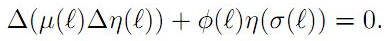Our oscillation results improve and extend the earlier ones. Examples illustrating the results are provided.

4: Paper Source PDF document

Paper's Title:

On Sandwich Theorems for Certain Subclass of Analytic Functions Involving Dziok-Srivastava Operator

Author(s):

T. N. Shanmugam, M. P. Jeyarama and A. Singaravelu

Department of Mathematics
College of Engineering, Anna University
Chennai - 600 025,
India
drtns2001@yahoo.com

Department of Mathematics
Easwari Engineering College
Ramapuram, Chennai - 600089
jeyaraman-mp@yahoo.co.i

Department of Mathematics
Valliammai Engineering College
Chennai - 603203
asing-59@yahoo.com

Abstract:

The purpose of this present paper is to derive some subordination and superordination results for certain normalized analytic functions in the open unit disk, acted upon by Dziok-Srivastava operator. Relevant connections of the results, which are presented in this paper, with various known results are also considered.

4: Paper Source PDF document

Paper's Title:

The Invariant Subspace Problem for Linear Relations on Hilbert Spaces

Author(s):

Daniel Grixti-Cheng

Department of Mathematics and Statistics,
The University of Melbourne,
Melbourne, VIC, 3010
Australia.
D.Grixti@ms.unimelb.edu.au

Abstract:

We consider the invariant subspace problem for linear relations on Hilbert spaces with the aim of promoting interest in the problem as viewed from the theory of linear relations. We present an equivalence between the single valued and multivalued invariant subspace problems and give some new theorems pertaining to the invariant subspace problem for linear relations on a Hilbert space.

4: Paper Source PDF document

Paper's Title:

Some Inequalities for a Certain Class of Multivalent Functions Using Multiplier Transformation

Author(s):

Department Of Applied Mathematics
Sri Venkateswara College Of Engineering
Sriperumbudur, Chennai - 602105,
India.
suchithravenkat@yahoo.co.in

Department Of Mathematics,
Chennai - 600059,
India.

Department Of Applied Mathematics
Sri Venkateswara College Of Engineering
Sriperumbudur, Chennai - 602105,
India.
ganga@svce.ac.in

Department Of Mathematics,
Easwari Engineering College
Ramapuram, Chennai - 600089,
India.
ganga@svce.ac.in

Abstract:

The object of the present paper is to derive several inequalities associated with differential subordinations between analytic functions and a linear operator defined for a certain family of p-valent functions, which is introduced here by means of a family of extended multiplier transformations. Some special cases and consequences of the main results are also considered.

4: Paper Source PDF document

Paper's Title:

Neighborhoods of Certain Subclasses of Analytic Functions of Complex Order with Negative Coefficients

Author(s):

Department of Applied Mathematics,
Sri Venkateswara College of Engineering,
Sriperumbudur, Chennai - 602105,
India.

Department of Mathematics,
Chennai - 600059,
India

Department of Applied Mathematics,
Sri Venkateswara College of Engineering,
Sriperumbudur, Chennai - 602105,
India.

Department of Mathematics,
Easwari Engineering College,
Ramapuram, Chennai - 600089,
India

Abstract:

The main object of this paper is to prove several inclusion relations associated with the (n, δ) neighborhoods of various subclasses of convex functions of complex order by making use of the known concept of neighborhoods of analytic functions.

4: Paper Source PDF document

Paper's Title:

Generalized Efficient Solutions to One Class of Vector Optimization Problems in Banach Space

Author(s):

Peter I. Kogut, Rosanna Manzo, and Igor V. Nechay

Department of Differential Equations,
Dnipropetrovsk National University,
Naukova str., 13, 49050 Dnipropetrovsk,
Ukraine
p.kogut@i.ua

Dipartimento di Ingegneria Dell’informazione e Matematica Applicata,
Universitŕ di Salerno,
Via Ponte Don Melillo, 84084 Fisciano (Sa),
Italy
manzo@diima.unisa.it

Department of Technical Cybernetics,
Dnipropetrovsk Technical University,
Dnipro
petrovsk,
Ukraine
i.nechay@i.ua

Abstract:

In this paper, we study vector optimization problems in Banach spaces for essentially nonlinear operator equations with additional control and state constraints. We assume that an objective mapping possesses a weakened property of lower semicontinuity and make no assumptions on the interior of the ordering cone. Using the penalization approach we derive both sufficient and necessary conditions for the existence of efficient solutions of the above problems. We also prove the existence of the so-called generalized efficient solutions via the scalarization of some penalized vector optimization problem.

4: Paper Source PDF document

Paper's Title:

Equivalence of the Nonsmooth Nonlinear Complementarity Problems to Unconstrained Minimization

Author(s):

M. A. Tawhid and J. L. Goffin

Department of Mathematics and Statistics, School of Advanced Technologies and Mathematics,
Thompson Rivers University,
900 McGill Road, PO Box 3010, Kamloops, BC V2C 5N3
Alexandria University and Egypt Japan University of Science and Technology,
Alexandria-Egypt
mtawhid@tru.ca

Faculty of Management, McGill University,
1001 Sherbrooke Street West, Montreal, Quebec, H3A 1G5
Jean-Louis.Goffin@McGill.ca

Abstract:

This paper deals with nonsmooth nonlinear complementarity problem, where the underlying functions are nonsmooth which admit the H-differentiability but not necessarily locally Lipschitzian or directionally differentiable. We consider a reformulation of the nonlinear complementarity problem as an unconstrained minimization problem. We describe H-differentials of the associated penalized Fischer-Burmeister and Kanzow and Kleinmichel merit functions. We show how, under appropriate P0, semimonotone (E0), P, positive definite, and strictly semimonotone (E) -conditions on an H-differential of f, finding local/global minimum of a merit function (or a `stationary point' of a merit function) leads to a solution of the given nonlinear complementarity problem. Our results not only give new results but also unify/extend various similar results proved for C1.

4: Paper Source PDF document

Paper's Title:

C*-valued metric projection and Moore-Penrose inverse on Hilbert C*-modules

Author(s):

M. Eshaghi Gordji, H. Fathi and S.A.R. Hosseinioun

Department of Mathematics,
Semnan University, P.O. Box 35195-363, Semnan,
Iran.
Center of Excellence in Nonlinear Analysis and Applications (CENAA),
Semnan University,
Iran.

Department of Mathematics,
Shahid Beheshti University, Tehran,
Iran.
E-mail: Hedayat.fathi@yahoo.com

Department of Mathematical Sciences,
University of Arkansas, Fayetteville, Arkansas 72701,
USA.
E-mail: shossein@uark.net

Abstract:

Let t be a regular operator between Hilbert C*-modules and t be its Moore-Penrose inverse. We give some characterizations for t based on C*-valued metric projection. Moore-Penrose inverse of bounded operators and elements of a C*-algebra is studied as a special case.

4: Paper Source PDF document

Paper's Title:

Credibility Based Fuzzy Entropy Measure

Author(s):

G. Yari, M. Rahimi, B. Moomivand and P. Kumar

Department of Mathematics,
Iran University of Science and Technology,
Tehran,
Iran.
E-mail: Yari@iust.ac.ir
E-mail: Mt_Rahimi@iust.ac.ir
URL: http://www.iust.ac.ir/find.php?item=30.11101.20484.en
URL: http://webpages.iust.ac.ir/mt_rahimi/en.html

Qarzol-hasaneh
Mehr Iran Bank, Tehran,
Iran.
E-mail: B.moomivand@qmb.ir

Department of Mathematics and Statistics,
University of Northern British Columbia,
Prince George, BC,
E-mail: Pranesh.Kumar@unbc.ca

Abstract:

Fuzzy entropy is the entropy of a fuzzy variable, loosely representing the information of uncertainty. This paper, first examines both previous membership and credibility based entropy measures in fuzzy environment, and then suggests an extended credibility based measure which satisfies mostly in Du Luca and Termini axioms. Furthermore, using credibility and the proposed measure, the relative entropy is defined to measure uncertainty between fuzzy numbers. Finally we provide some properties of this Credibility based fuzzy entropy measure and to clarify, give some examples.

4: Paper Source PDF document

Paper's Title:

Composite Variational-Like Inequalities Given By Weakly Relaxed ζ-Semi-Pseudomonotone Multi-Valued Mapping

Author(s):

Syed Shakaib Irfan, Iqbal Ahmad, Zubair Khan and Preeti Shukla

College of Engineering, Qassim University
Buraidah, Al-Qassim,
Saudi Arabia.
E-mail: shakaib@qec.edu.sa

College of Engineering, Qassim University
Buraidah, Al-Qassim,
Saudi Arabia.
E-mail: iqbal@qec.edu.sa

Department of Mathematics,
Integral University Lucknow,
India.
E-mail: zkhan@iul.ac.in

Department of Mathematics,
Integral University Lucknow,
India.
E-mail: shuklapreeti1991@gmail.com

Abstract:

In this article, we introduce a composite variational-like inequalities with weakly relaxed ζ-pseudomonotone multi-valued maping in reflexive Banach spaces. We obtain existence of solutions to the composite variational-like inequalities with weakly relaxed ζ-pseudomon -otone multi-valued maps in reflexive Banach spaces by using KKM theorem. We have also checked the solvability of the composite variational-like inequalities with weakly relaxed ζ-semi-pseudomonotone multi-valued maps in arbitrary Banach spaces using Kakutani-Fan-Glicksberg fixed point theorem.

4: Paper Source PDF document

Paper's Title:

Numerical Approximation by the Method of Lines with Finite-volume Approach of a Solute Transport Equation in Periodic Heterogeneous Porous Medium

Author(s):

D. J. Bambi Pemba and B. Ondami

Université Marien Ngouabi,
Factuté des Sciences et Techniques,
BP 69, Brazzaville,
Congo.
E-mail: bondami@gmail.com

Abstract:

In this paper we are interested in the numerical approximation of a two-dimensional solute transport equation in heterogeneous porous media having periodic structures. It is a class of problems which has been the subject of various works in the literature, where different methods are proposed for the determination of the so-called homogenized problem. We are interested in this paper, in the direct resolution of the problem, and we use the method of lines with a finite volume approach to discretize this equation. This discretization leads to an ordinary differential equation (ODE) that we discretize by the Euler implicit scheme. Numerical experiments comparing the obtained solution and the homogenized problem solution are presented. They show that the precision and robustness of this method depend on the ratio between, the mesh size and the parameter involved in the periodic homogenization.

4: Paper Source PDF document

Paper's Title:

Jordan Canonical Form of Interval Matrices and Applications

Author(s):

S. Hema Surya, T. Nirmala and K. Ganesan

Department of Mathematics, College of Engineering and Technology,
SRM Institute of Science and Technology,
Kattankulathur,
Chennai-603203,
India.
E-mail: nirmalat@srmist.edu.in
URL: https://www.srmist.edu.in/faculty/dr-t-nirmala/

Abstract:

A square interval matrix over R can be converted to diagonal form if certain prerequisites are satisfied. However not all square matrices can be diagonalized. As a consequence, we strive the next simplest form to which it can be reduced while retaining important properties such as eigenvalues, rank, nullity, and so on. It turns out that any real interval matrix has a Jordan Canonical Form (JCF) over E if it has n interval eigenvalues in IR. We discuss in this paper a method for computing the Jordan canonical form of an interval matrix using a new pairing technique and a new type of interval arithmetic that will make classifying and analyzing interval matrices easier and more efficient. We conclude with a numerical example that supports the theory and application of predator-prey model.

3: Paper Source PDF document

Paper's Title:

An alternative proof of monotonicity for the extended mean values

Author(s):

Chao-Ping Chen and Feng Qi

Department of Applied Mathematics and Informatics, Research Institute of Applied Mathematics,
Henan Polytechnic University, Jiaozuo City, Henan 454000, China

chenchaoping@hpu.edu.cn

Department of Applied Mathematics and Informatics, Research Institute of Applied Mathematics,
Henan Polytechnic University, Jiaozuo City, Henan 454000, China

qifeng@hpu.edu.cn, fengqi618@member.ams.org
Url
: http://rgmia.vu.edu.au/qi.html, http://dami.hpu.edu.cn/qifeng.html

Abstract:

An alternative proof of monotonicity for the extended mean values is given.

3: Paper Source PDF document

Paper's Title:

Two Mappings Related to Steffensen's Inequalities

Author(s):

Liang-Cheng Wang

School of Mathematical Science,
Chongqing Institute of Technology,
Xingsheng Lu 4,
Yangjiaping 400050, Chongqing City,
China.
wangliangcheng@163.com

Abstract:

In this paper, we define two mappings closely connected with Steffensen's inequalities, investigate their main properties, give some refinements for Steffensen's inequalities and obtain new inequalities.

3: Paper Source PDF document

Paper's Title:

A New Hardy-Hilbert's Type Inequality for Double Series and its Applications

Author(s):

Mingzhe Gao

Department of Mathematics and Computer Science, Normal College Jishou University,
Jishou Hunan, 416000,
People's Republic of China
mingzhegao1940@yahoo.com.cn

Abstract:

In this paper, it is shown that a new Hardy-Hilbert’s type inequality for double series can be established by introducing a parameter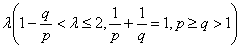and the weight function of the form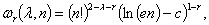where c is Euler constant and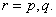And the coefficient is proved to be the best possible. And as the mathematics aesthetics, several important constants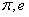and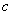appear simultaneously in the coefficient and the weight function when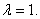In particular, for case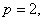some new Hilbert’s type inequalities are obtained. As applications, some extensions of Hardy-Littlewood’s inequality are given.

3: Paper Source PDF document

Paper's Title:

A Coefficient Inequality For Certain Subclasses of Analytic Functions Related to Complex Order

Author(s):

B. Srutha Keerthi, B. Adolf Stephen and S. Sivasubramanian

Department Of Applied Mathematics, Sri Venkateswara College Of Engineering, Anna University,
Sriperumbudur, Chennai - 602 105,
India.
laya@svce.ac.in

Department of Mathematics, Madras Christian College, Chennai - 600059,
India

Department of Mathematics, College of Engineering, Anna University,
India.
sivasaisastha@rediffmail.com

Abstract:

In this present investigation, the authors obtain coefficient inequality for certain normalized analytic functions of complex order f(z) defined on the open unit disk for which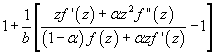(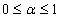and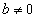be a complex number) lies in a region starlike with respect to 1 and is symmetric with respect to the real axis. Also certain applications of the main result for a class of functions of complex order defined by convolution are given. As a special case of this result, coefficient inequality for a class of functions defined through fractional derivatives is obtained. The motivation of this paper is to give a generalization of the coefficient inequalities of the subclasses of starlike and convex functions of complex order.

3: Paper Source PDF document

Paper's Title:

On the Generalized Inverse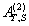over Integral Domains

Author(s):

Yaoming Yu and Guorong Wang

College of Education, Shanghai Normal University
Shanghai 200234
People's Republic of China.
yuyaoming@online.sh.cn
grwang@shnu.edu.cn

Abstract:

In this paper, we study further the generalized inverseof a matrix A over an integral domain. We give firstly some necessary and sufficient conditions for the existence of the generalized inverse, an explicit expression for the elements of the generalized inverseand an explicit expression for the generalized inverse, which reduces to the {1} inverse. Secondly, we verify that the group inverse, the Drazin inverse, the Moore-Penrose inverse and the weighted Moore-Penrose inverse are identical with the generalized inverse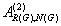for an appropriate matrix G, respectively, and then we unify the conditions for the existence and the expression for the elements of the weighted Moore-Penrose inverse, the Moore-Penrose inverse, the Drazin inverse and the group inverse over an integral domain. Thirdly, as a simple application, we give the relation between some rank equation and the existence of the generalized inverse, and a method to compute the generalized inverse. Finally, we give an example of evaluating the elements ofwithout calculating.

3: Paper Source PDF document

Paper's Title:

Two Classes of Completely Monotonic Functions Involving Gamma and Polygamma Functions

Author(s):

Bai-Ni Guo, Xiao-Ai Li and Feng Qi

School of Mathematics and Informatics, Henan Polytechnic University,
Jiaozuo City, Henan Province, 454010,
China.
bai.ni.guo@gmail.com

College of Mathematics and Information Science,
Henan Normal University, Xinxiang City,
Henan Province, 453007,
China.
lxa.hnsd@163.com

Research Institute of Mathematical Inequality Theory,
Henan Polytechnic University, Jiaozuo City,
Henan Province, 454010,
China
qifeng618@gmail.com
qifeng618@hotmail.com
qifeng618@qq.com
URLhttp://rgmia.vu.edu.au/qi.html

Abstract:

The function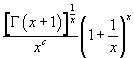is logarithmically completely monotonic in (0,∞) if and only if c≥1 and its reciprocal is logarithmically completely monotonic in (0,∞) if and only if c≤0. The function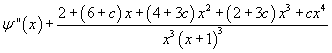is completely monotonic in (0,∞) if and only if c≥1 and its negative is completely monotonic in (0,∞)  if and only if c≤0.

3: Paper Source PDF document

Paper's Title:

A Double Inequality for Divided Differences and Some Identities of the Psi and Polygamma Functions

Author(s):

B. N. Guo and F. Qi

School of Mathematics and Informatics, Henan Polytechnic University, Jiaozuo City, Henan Province, 454010, China
bai.ni.guo@gmail.com, bai.ni.guo@hotmail.com

School of Research Institute of Mathematical Inequality Theory, Henan Polytechnic University, Jiaozuo City, Henan Province, 454010, China
qifeng618@gmail.com, qifeng618@hotmail.com, qifeng618@qq.com
URL:http://qifeng618.spaces.live.com

Abstract:

In this short note, from the logarithmically completely monotonic property of the function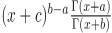, a double inequality for the divided differences and some identities of the psi and polygamma functions are presented.

3: Paper Source PDF document

Paper's Title:

Hyperbolic Barycentric Coordinates

Author(s):

Abraham A. Ungar

Department of Mathematics, North Dakota State University,
Fargo, ND 58105,
USA
Abraham.Ungar@ndsu.edu
URL
: http://math.ndsu.nodak.edu/faculty/ungar/

Abstract:

A powerful and novel way to study Einstein's special theory of relativity and its underlying geometry, the hyperbolic geometry of Bolyai and Lobachevsky, by analogies with classical mechanics and its underlying Euclidean geometry is demonstrated. The demonstration sets the stage for the extension of the notion of barycentric coordinates in Euclidean geometry, first conceived by Möbius in 1827, into hyperbolic geometry. As an example for the application of hyperbolic barycentric coordinates, the hyperbolic midpoint of any hyperbolic segment, and the centroid and orthocenter of any hyperbolic triangle are determined.

3: Paper Source PDF document

Paper's Title:

Hyperbolic Models Arising in the Theory of Longitudinal Vibration of Elastic Bars

Author(s):

1I. Fedotov, 1J. Marais, 1,2M. Shatalov and 1H.M. Tenkam

1Department of Mathematics and Statistics,
Tshwane University of Technology
Private Bag X6680, Pretoria 0001
South Africa.

fedotovi@tut.ac.za, julian.marais@gmail.com, djouosseutenkamhm@tut.ac.za.

2Manufacturing and Materials
Council of Scientific and Industrial Research (CSIR)
P.O. Box 395, Pretoria, 0001
South Africa.
mshatlov@csir.co.za

Abstract:

In this paper a unified approach to the derivation of families of one
dimensional hyperbolic differential equations and boundary conditions describing
the longitudinal vibration of elastic bars is outlined. The longitudinal and
lateral displacements are expressed in the form of a power series expansion in
the lateral coordinate. Equations of motion and boundary conditions are derived
using Hamilton's variational principle. Most of the well known models in this
field fall within the frames of the proposed theory, including the classical
model, and the more elaborated models proposed by by Rayleigh, Love, Bishop,
Mindlin, Herrmann and McNiven. The exact solution is presented for the
Mindlin-Herrmann case in terms of Green functions. Finally, deductions regarding
the accuracy of the models are made by comparison with the exact
Pochhammer-Chree solution for an isotropic cylinder.

3: Paper Source PDF document

Paper's Title:

Existence of Positive Solutions for Nonlinear Fractional Differential Equations with Multi-point Boundary Conditions

Author(s):

Khenchela University, Department of Mathematics,
Khenchela, 40000,
Algeria.

Abstract:

This paper is devoted to the existence results of positive solutions for a nonlinear fractional differential equations with multi-point boundary conditions. By means of the Schauder fixed point theorem, some results on the existence are obtained.

3: Paper Source PDF document

Paper's Title:

Local Boundedness of Weak Solutions for Singular Parabolic Systems of p-Laplacian Type

Author(s):

Corina Karim, Marjono

Department of Mathematics,
Universitas Brawijaya,
Indonesia.
E-mail:  co_mathub@ub.ac.id, marjono@ub.ac.id

Abstract:

We study the local boundedness of weak solutions for evolutional p-Laplacian systems in the singular case. The initial data is belonging to Lebesgue space L (0,T;W(1,p) (Ω,Rn )). We use intrinsic scaling method to treat the boundedness of weak solutions. The main result is to make the local boundedness of weak solution for the systems well-worked in the intrinsic scaling.

3: Paper Source PDF document

Paper's Title:

Dynamical Analysis of HIV/AIDS Epidemic Model with Two Latent Stages, Vertical Transmission and Treatment

Author(s):

Nur Shofianah, Isnani Darti, Syaiful Anam

Mathematics Department,Faculty of Mathematics and Natural Sciences.
University of Brawijaya,
Jl. Veteran, Malang 65145,
Indonesia.
E-mail: nur_shofianah@ub.ac.id, isnanidarti@ub.ac.id, syaiful@ub.ac.id

Abstract:

We discuss about dynamical analysis of HIV/AIDS epidemic model with two latent stages, vertical transmission and treatment. In this model, the spreading of HIV occurs through both horizontal and vertical transmission. There is also treatment for individual who has been HIV infected. The latent stage is divided into slow and fast latent stage based on the immune condition which varies for each individual. Dynamical analysis result shows that the model has two equilibrium points: the disease-free equilibrium point and the endemic equilibrium point. The existence and global stability of equilibrium points depend on the basic reproduction number R0. When R0 <1, only the disease-free equilibrium point exists. If R0 >1, there are two equilibrium points, which are the disease-free equilibrium point and the endemic equilibrium point. Based on the result of stability analysis, the disease-free equilibrium point is globally asymptotically stable if R0 <1, while if R0 > 1 and p=q, the endemic equilibrium point will be globally asymptotically stable. In the end, we show some numerical simulations to support the analytical result.

3: Paper Source PDF document

Paper's Title:

A Low Order Least-Squares Nonconforming Finite Element Method for Steady Magnetohydrodynamic Equations

Author(s):

Z. Yu, D. Shi and H. Zhu

College of Science,
Zhongyuan University of Technology,
Zhengzhou 450007,
China.
E-mail: 5772@zut.edu.cn

School of Mathematics and Statistics,
Zhengzhou University,
Zhengzhou 450001,
China.
E-mail: shi_dy@126.com

Mathematics Department,
University of Southern Mississippi,
Hattiesburg MS, 39406,
U.S.A
E-mail: huiqing.zhu@usm.edu

Abstract:

A low order least-squares nonconforming finite element (NFE) method is proposed for magnetohydrodynamic equations with EQ1rot element and zero-order Raviart-Thomas element. Based on the above element's typical interpolations properties, the existence and uniqueness of the approximate solutions are proved and the optimal order error estimates for the corresponding variables are derived.

3: Paper Source PDF document

Paper's Title:

Global Analysis on Riemannian Manifolds

Author(s):

Louis Omenyi and Michael Uchenna

Department of Mathematics, Computer Science, Statistics and Informatics,
Alex Ekwueme Federal University, Ndufu-Alike,
Nigeria.
E-mail: omenyi.louis@funai.edu.ng, michael.uchenna@funai.edu.ng
URL: http://www.funai.edu.ng

Abstract:

In this paper, an exposition of the central concept of global analysis on a Riemannan manifold is given. We extend the theory of smooth vector fields from open subsets of Euclidean space to Riemannan manifolds. Specifically, we prove that a Riemannian manifold admits a unique solution for a system of ordinary differential equations generated by the flow of smooth tangent vectors. The idea of partial differential equations on Riemannian manifold is highlighted on the unit sphere.

3: Paper Source PDF document

Paper's Title:

Attempts to Define a Baum--Connes Map Via Localization of Categories for Inverse Semigroups

Author(s):

Bernhard Burgstaller

Departamento de Matematica,
CEP 88.040-900 Florianopolis-SC,
Brasil.
E-mail: bernhardburgstaller@yahoo.de
URL: http://mathematik.work/bernhardburgstaller/index.html

Abstract:

An induction functor in inverse semigroup equivariant KK-theory is considered, and together with %a restriction functors certain results similar to those known from the Mackey machinery are shown. It is also verified that for any so-called E-continuous inverse semigroup its equivariant KK-theory satisfies the universal property and is a triangulated category.

3: Paper Source PDF document

Paper's Title:

Fractional exp(-φ(ξ))- Expansion Method and its Application to Space--Time Nonlinear Fractional Equations

Author(s):

A. A. Moussa and L. A. Alhakim

Department of Management Information System and Production Management,
College of Business and Economics, Qassim University,
P.O. BOX 6666, Buraidah: 51452,
Saudi Arabia.
E-mail: Alaamath81@gmail.com

Department of Management Information System and Production Management,
College of Business and Economics, Qassim University,
P.O. BOX 6666, Buraidah: 51452,
Saudi Arabia.
E-mail: Lama2736@gmail.com

Abstract:

In this paper, we mainly suggest a new method that depends on the fractional derivative proposed by Katugampola for solving nonlinear fractional partial differential equations. Using this method, we obtained numerous useful and surprising solutions for the space--time fractional nonlinear Whitham--Broer--Kaup equations and space--time fractional generalized nonlinear Hirota--Satsuma coupled KdV equations. The solutions obtained varied between hyperbolic, trigonometric, and rational functions, and we hope those interested in the real-life applications of the previous two equations will find this approach useful.

3: Paper Source PDF document

Paper's Title:

Inequalities of Gamma Function Appearing in Generalizing Probability Sampling Design

Author(s):

Department of Mathematics,
Yarmouk University,
Irbid 21163,
Jordan.

Department of Statistics,
Yarmouk University,
Irbid 21163,
Jordan.

Abstract:

In this paper, we investigate the complete monotonicity of some functions involving gamma function. Using the monotonic properties of these functions, we derived some inequalities involving gamma and beta functions. Such inequalities can be used to generalize different probability distribution functions. Also, they can be used to generalize some statistical designs, e.g., the probability proportional to the size without replacement design.

3: Paper Source PDF document

Paper's Title:

Construction of a Frame Multiresolution Analysis on Locally Compact Abelian Groups

Author(s):

R. Kumar and Satyapriya

Department of Mathematics,
Kirori Mal College,
University of Delhi,
Delhi,
India.
E-mail: rajkmc@gmail.com

Department of Mathematics,
University of Delhi,
Delhi,
India.
E-mail: kmc.satyapriya@gmail.com

Abstract:

The frame multiresolution analysis (FMRA) on locally compact Abelian groups has been studied and the results concerning classical MRA have been worked upon to obtain new results. All the necessary conditions, which need to be imposed on the scaling function φ to construct a wavelet frame via FMRA, have been summed up. This process of construction of FMRA has aptly been illustrated by sufficient examples.

3: Paper Source PDF document

Paper's Title:

Improved Oscillation Criteria of Second-Order Advanced Non-canonical Difference Equation

Author(s):

G. E. Chatzarakis1, N. Indrajith2, S. L. Panetsos1, E. Thandapani3

1Department of Electrical and Electronic Engineering Educators
School of Pedagogical and Technological Education,
Marousi 15122, Athens,
Greece.
E-mail: gea.xatz@aspete.gr, geaxatz@otenet.gr
spanetsos@aspete.gr

2Department of Mathematics,
Presidency College, Chennai - 600 005,
India.
E-mail: indrajithna@gmail.com

3Ramanujan Institute for Advanced Study in Mathematics,
University of Madras Chennai - 600 005,
India.
E-mail: ethandapani@yahoo.co.in

Abstract:

Employing monotonic properties of nonoscillatory solutions, we derive some new oscillation criteria for the second-order advanced non-canonical difference equation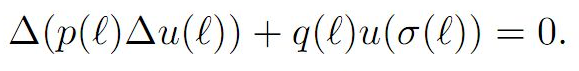Our results extend and improve the earlier ones. The outcome is illustrated via some particular difference equations.

3: Paper Source PDF document

Paper's Title:

Fekete-Szegö Inequality for Sakaguchi Type of functions in Petal Shaped Domain

Author(s):

E. K. Nithiyanandham and B. Srutha Keerthi

Division of Mathematics, School of Advanced Sciences,
Vellore Institute of Technology Chennai Campus,
Chennai - 600 048,
India.
E-mail: nithiyankrish@gmail.com

Division of Mathematics, School of Advanced Sciences,
Vellore Institute of Technology Chennai Campus,
Chennai - 600 048,
India.
E-mail: keerthivitmaths@gmail.com

Abstract:

In this paper, we estimate coefficient bounds,|a_2|,|a_3| and |a_4|, Fekete-Szegö inequality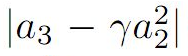and Toeplitz determinant T2(2) and T3(1) for functions belonging to the following class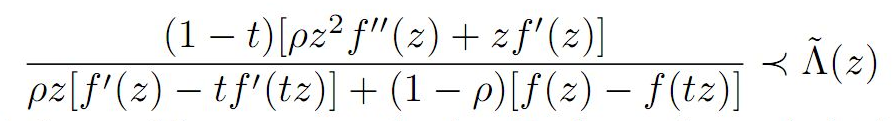the function being holomorphic, we expand using Taylor series and obtain several corollaries and consequences for the main result.

3: Paper Source PDF document

Paper's Title:

Toeplitz Determinant for Sakaguchi Type Functions Under Petal Shaped Domain

Author(s):

B. Nandhini and B. Srutha Keerthi

Division of Mathematics, School of Advanced Sciences,
Vellore Institute of Technology Chennai Campus,
Chennai - 600 048,
India.

Division of Mathematics, School of Advanced Sciences,
Vellore Institute of Technology Chennai Campus,
Chennai - 600 048,
India.
E-mail: keerthivitmaths@gmail.com

Abstract:

We introduce a new general subclass GPt,ρ of Sakaguchi kind function on a Petal shaped domain. We obtain coefficients bounds and upper bounds for the Fekete-Szegö functional over the class. From these functions we obtain the bounds of first four coefficients, and then we have derived the Toeplitz determinant T2(2) and T3(1) whose diagonal entries are the coefficients of functions.

2: Paper Source PDF document

Paper's Title:

Monotonicity Properties for Generalized Logarithmic Means

Author(s):

Chao-Ping Chen and Feng Qi

Department of Applied Mathematics and Informatics, Research Institute of Applied Mathematics,
Henan Polytechnic University, Jiaozuo City, Henan 454000, China

chenchaoping@hpu.edu.cn

Department of Applied Mathematics and Informatics, Research Institute of Applied Mathematics,
Henan Polytechnic University, Jiaozuo City, Henan 454000, China

qifeng@hpu.edu.cn, fengqi618@member.ams.org

U
rl: http://rgmia.vu.edu.au/qi.html, http://dami.hpu.edu.cn/qifeng.html

Abstract:

In this paper, we consider the monotonicity properties for ratio of two generalized logarithmic means, and then use it to extend and complement a recently published result of F. Qi and B.-N. Guo.

2: Paper Source PDF document

Paper's Title:

Reverse of Martin's Inequality

Author(s):

Chao-Ping Chen, Feng Qi, and Sever S. Dragomir

Department of Applied Mathematics and Informatics,
Research Institute of Applied Mathematics,
Henan Polytechnic University,
Jiaozuo City, Henan 454010, China
chenchaoping@sohu.com; chenchaoping@hpu.edu.cn

Department of Applied Mathematics and Informatics,
Research Institute of Applied Mathematics,
Henan Polytechnic University,
Jiaozuo City, Henan 454010, China
qifeng@hpu.edu.cn
Url: http://rgmia.vu.edu.au/qi.html

School of Computer Science and Mathematics,
Victoria University of Technology,
P. O. Box 14428, Melbourne City Mc,
Victoria 8001, Australia
Sever.Dragomir@vu.edu.au
Url: http://rgmia.vu.edu.au/SSDragomirWeb.html

Abstract:

In this paper, it is proved that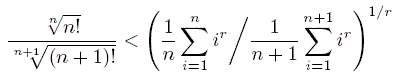for all natural numbers n, and all real r < 0.

2: Paper Source PDF document

Paper's Title:

On the Hohov Convolution Of The Class Sp(α,β)

Author(s):

T. N. Shanmugam and S. Sivasubramanian

Department of Mathematics,
Anna University,
Chennai 600025,
shan@annauniv.edu

Department of Mathematics,
Easwari Engineering College,
Chennai-600089,
sivasaisastha@rediffmail.com

Abstract:

Let F(a,b;c;z) be the Gaussian hypergeometric function and Ia,b;c(f)=zF(a,b;c;z)*f(z) be the Hohlov operator defined on the class A of all normalized analytic functions. We determine conditions on the parameters a,b,c such that Ia,b;c(f) will be in the class of parabolic starlike functions Sp(α,β). Our results extend several earlier results.

2: Paper Source PDF document

Paper's Title:

Positive Periodic Time-Scale Solutions for Functional Dynamic Equations

Author(s):

Douglas R. Anderson and Joan Hoffacker

Department of Mathematics and Computer Science
Concordia College
andersod@cord.edu
URL:
http://www.cord.edu/faculty/andersod/

Department of Mathematical Sciences
Clemson University
Clemson, SC 29634 USA
johoff@clemson.edu
URL:
http://www.math.clemson.edu/facstaff/johoff.htm

Abstract:

Using Krasnoselskii's fixed point theorem, we establish the existence of positive periodic solutions to two pairs of related nonautonomous functional delta dynamic equations on periodic time scales, and then extend the discussion to higher-dimensional equations. Two pairs of corresponding nabla equations are also provided in an analogous manner.

2: Paper Source PDF document

Paper's Title:

Differential Sandwich Theorems for Some Subclasses of Analytic Functions

Author(s):

T. N. Shanmugam, V. Ravichandran and S. Sivasubramanian

Department of Mathematics, College of Engineering,
Anna university, Chennai 600 025,
India
shan@annauniv.edu
URL: http://www.annauniv.edu/shan

School of Mathematical Sciences,
Universiti Sains Malaysia,
11800 USM Penang,
Malaysia
vravi@cs.usm.my
URL: http://cs.usm.my/~vravi

Department of Mathematics, Easwari Engineering college,
Ramapuram, Chennai 600 089,
India
sivasaisastha@rediffmail.com

Abstract:

Let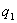and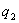be univalent in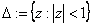with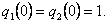We give some applications of first order differential subordination and superordination to obtain sufficient conditions for normalized analytic function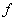with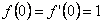to satisfy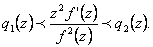2: Paper Source PDF document

Paper's Title:

Uniqueness of Meromorphic Functions that Share Three Values

Author(s):

Abhijit Banerjee

Department of Mathematics
Kalyani Government Engineering College
West Bengal 741235
India.
abanerjee_kal@yahoo.co.in
abanerjee@mail15.com
abanerjee_kal@rediffmail.com

Abstract:

In the paper dealing with the uniqueness problem of meromorphic functions we prove five theorems one of which will improve a result given by Lahiri \cite{5} and the remaining will supplement some previous results.

2: Paper Source PDF document

Paper's Title:

On the Fekete-Szegő Inequality for Some Subclasses of Analytic Functions

Author(s):

T.N. Shanmugam and A. Singaravelu

Department of Mathematics,
College of Engineering,
Anna University, Chennai-600 025,
shan@annauniv.edu

Department of Mathematics,
Valliammai Engineering College,
Chennai-603 203,
sivasaisastha@rediffmail.com

Abstract:

In this present investigation, the authors obtainFekete-Szegő's inequality for certain normalized analytic functions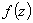defined on the open unit disk for which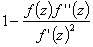lie in a region starlike with respect to 1 and symmetric with respect to the real axis. Also certain applications of the main result for a class of functions defined by convolution are given. As a special case of this result, Fekete-Szegő's inequality for a class of functions defined through fractional derivatives is also obtained.

2: Paper Source PDF document

Paper's Title:

A Strengthened Hardy-Hilbert's Type Inequality

Author(s):

Weihong Wang and Bicheng Yang

Department of Mathematics, Guangdong Education Institute,
Guangzhou, Guangdong 520303,
People's Republic Of China
wwh@gdei.edu.cn
bcyang@pub.guangzhou.gd.cn
URL: http://www1.gdei.edu.cn/yangbicheng/index.html

Abstract:

By using the improved Euler-Maclaurin's summation formula and estimating the weight coefficient, we give a new strengthened version of the more accurate Hardy-Hilbert's type inequality. As applications, a strengthened version of the equivalent form is considered.

2: Paper Source PDF document

Paper's Title:

On Some Ramanujan's Schläfli Type Modular Equations

Author(s):

K. R. Vasuki

Department of Mathematics, Acharya Institute of Technology, Soldevanahalli,
Chikkabanavara (Post), Hesaragatta Main Road, Bangalore-560 090,
INDIA.
vasuki_kr@hotmail.com

Abstract:

In this paper, we give new proof of certain Ramanujan-Schläfli modular equations. We also obtain a new modular equation of degree 23.

2: Paper Source PDF document

Paper's Title:

On Positive Entire Solutions of Second Order Quasilinear Elliptic Equations

Author(s):

Zuodong Yang and Honghui Yin

Institute of Mathematics, School of Mathematics and Computer Science,
Nanjing Normal University, Jiangsu Nanjing 210097,
China;
zdyang_jin@263.net

Department of Mathematics, Huaiyin Teachers College,
Jiangsu Huaian 223001,
China;
School of Mathematics and Computer Sciences,
Nanjing Normal University, Jiangsu Nanjing 210097,
China.
yin_hh@sina.com

Abstract:

In this paper, our main purpose is to establish the existence theorem of positive entire solutions of second order quasilinear elliptic equations under new conditions. The main results of the present paper are new and extend the previously known results.

2: Paper Source PDF document

Paper's Title:

Komatu Integral Transforms of Analytic Functions Subordinate to Convex Functions

Author(s):

T. N. Shanmugam and C. Ramachandran

Department of Mathematics, College of Engineering,
India
shan@annauniv.edu

Department of Mathematics, College of Engineering,
India
crjsp2004@yahoo.com

Abstract:

In this paper, we consider the class A of the functions f(z) of the form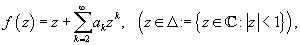which are analytic in an open disk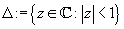and study certain subclass of the class A, for which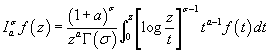has some property. Certain inclusion and the closure properties like convolution with convex univalent function etc. are studied.

2: Paper Source PDF document

Paper's Title:

Local and Global Existence and Uniqueness Results for Second and Higher Order Impulsive Functional Differential Equations with Infinite Delay

Author(s):

Johnny Henderson and Abdelghani Ouahab

Department of Mathematics, Baylor University,
Waco, Texas 76798-7328
USA.
Johnny_Henderson@baylor.edu

Laboratoire de Mathématiques, Université de Sidi Bel Abbés
BP 89, 22000 Sidi Bel Abbées,
Algérie.
ouahab@univ-sba.dz

Abstract:

In this paper, we discuss the local and global existence and uniqueness results for second and higher order impulsive functional differential equations with infinite delay. We shall rely on a nonlinear alternative of Leray-Schauder. For the global existence and uniqueness we apply a recent Frigon and Granas nonlinear alternative of Leray-Schauder type in Fréchet spaces.

2: Paper Source PDF document

Paper's Title:

A Nonlinear Proximal Alternating Directions Method for Structured Variational Inequalities

Author(s):

M. Li

Department of Management Science and Engineering, School of Economics and Management
Southeast University, Nanjing, 210096,
China.
liminnju@yahoo.com

Abstract:

In this paper, we present a nonlinear proximal alternating directions method (NPADM) for solving a class of structured variational inequalities (SVI). By choosing suitable Bregman functions, we generalize the proximal alternating directions method proposed by He, et al.. The convergence of the method is proved under quite mild assumptions and flexible parameter conditions.

2: Paper Source PDF document

Paper's Title:

A Subclass of Meromorphically Multivalent Functions with Applications to Generalized Hypergeometric Functions

Author(s):

M. K. Aouf

Mathematics Department, Faculty of Science,
Mansoura University 35516,
Egypt
mkaouf127@yahoo.com

Abstract:

In this paper a new subclass of meromorphically multivalent functions, which is defined by means of a Hadamard product (or convolution) involving some suitably normalized meromorphically p-valent functions. The main object of the present paper is to investigate the various important properties and characteristics of this subclass of meromorphically multivalent functions. We also derive many interesting results for the Hadamard products of functions belonging to this subclass. Also we consider several applications of our main results to generalized hypergeomtric functions.

2: Paper Source PDF document

Paper's Title:

Applications of Relations and Relators in the Extensions of Stability Theorems for Homogeneous and Additive Functions

Author(s):

Árpád Száz

Institute of Mathematics, University of Debrecen,
H-4010 Debrecen, Pf. 12,
Hungary
szaz@math.klte.hu

Abstract:

By working out an appropriate technique of relations and relators and extending the ideas of the direct methods of Z. Gajda and R. Ger, we prove some generalizations of the stability theorems of D. H. Hyers, T. Aoki, Th. M. Rassias and P. Găvruţă in terms of the existence and unicity of 2-homogeneous and additive approximate selections of generalized subadditive relations of semigroups to vector relator spaces. Thus, we obtain generalizations not only of the selection theorems of Z. Gajda and R. Ger, but also those of the present author.

2: Paper Source PDF document

Paper's Title:

On a Class of Uniformly Convex Functions Defined by Convolution with Fixed Coefficient

Author(s):

T. N. Shanmugam, S. Sivasubramanian, and G. Murugusundaramoorthy

Department of Mathematics,
College of Engineering,
Anna University,
Chennai - 600 025,
India.

drtns2001@yahoo.com

Department of Mathematics,
University College of Engineering,
Tindivanam
Anna University-Chennai,
Saram-604 703,
India.
sivasaisastha@rediffmail.com

School of Sciences
and Humanities,
VIT University, Vellore-632 014,
India.
gmsmoorthy@yahoo.com

Abstract:

We define a new subclass of uniformly convex functions with negative and fixed second coefficients defined by convolution. The main object of this paper is to obtain coefficient estimates distortion bounds, closure theorems and extreme points for functions belong to this new class . The results are generalized to families with fixed finitely many coefficients.

2: Paper Source PDF document

Paper's Title:

Two Remarks on Commutators of Hardy Operator

Author(s):

Yasuo Komori-Furuya

School of High Technology for Human Welfare
Tokai University
317 Nishino Numazu, Shizuoka 410-0395 Japan
komori@wing.ncc.u-tokai.ac.jp

Abstract:

Fu and Lu showed that
the commutator of multiplication operator by b and
the n-dimensional Hardy operator
is bounded on Lp if b is in some CMO space.
We shall prove the converse of this theorem
and also prove that their result is optimal by giving a counterexample

2: Paper Source PDF document

Paper's Title:

A New Property of General Means of Order p with an Application to the Theory of Economic Growth

Author(s):

Olivier de La Grandville

Department of Management Science and Engineering,
Huang Engineering Center, Stanford University,
475 Via Ortega, Stanford, California 94305
U.S.A.

lagrandvil@aol.com

Abstract:

The purpose of this note is to demonstrate a new property of the general mean of order p of m ordered positive numbers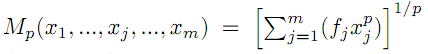. If p < 0 and if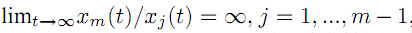, the elasticity of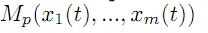with respect to xm, defined by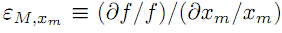, tends towards zero, and therefore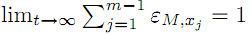. This property is then applied to optimal growth theory.

2: Paper Source PDF document

Paper's Title:

Solving Fractional Transport Equation via Walsh Function

Author(s):

L. M. F. N., Mathematics Department,
University of Setif,
Algeria
abdelouahak@yahoo.fr

Abstract:

In this paper we give a complete proof of A method for the solution of fractional transport equation in three-dimensional case by using Walsh function is presented. The main characteristic of this technique is that it reduces these problems to those of solving a system of algebraic equations, thus greatly simplifying the problem.

2: Paper Source PDF document

Paper's Title:

On Generalization of Hardy-type Inequalities

Author(s):

K. Rauf, S. Ponnusamy and J. O. Omolehin

Department of Mathematics,
University of Ilorin, Ilorin,
Nigeria
krauf@unilorin.edu.ng

Department of Mathematics,
Chennai- 600 036,
India
samy@iitm.ac.in

Department of Mathematics,
University of Ilorin, Ilorin,
Nigeria
omolehin_joseph@yahoo.com

Abstract:

This paper is devoted to some new generalization of Hardy-type integral inequalities and the reversed forms. The study is to determine conditions on which the generalized inequalities hold using some known hypothesis. Improvement of some inequalities are also presented.

2: Paper Source PDF document

Paper's Title:

Certain Coefficient Estimates for Bi-univalent Sakaguchi Type Functions

Author(s):

B. Srutha Keerthi, S. Chinthamani

Department of Applied Mathematics,
Sri Venkateswara College of Engineering,
Sriperumbudur, Chennai - 602105,
India

Abstract:

Estimates on the initial coefficients are obtained for normalized analytic functions f in the open unit disk with f and its inverse g = f-1 satisfying the conditions that zf'(z) / f(z) and zg'(z) / g(z) are both subordinate to a starlike univalent function whose range is symmetric with respect to the real axis. Several related classes of functions are also considered, and connections to earlier known results are made.

2: Paper Source PDF document

Paper's Title:

Sufficient Conditions for Certain Types of Functions to be Parabolic Starlike

Author(s):

Department of Mathematics,
Easwari Engineering College,
Ramapuram, Chennai - 89,
India.

Research Scholar,
Anna University,
Chennai

E-mail: ganga.megalai@gmail.com

E-mail: chinvicky@rediffmail.com

Abstract:

In this paper sufficient conditions are determined for functions of the form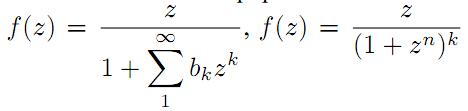and certain other types of functions to be parabolic starlike.

2: Paper Source PDF document

Paper's Title:

Some properties of quasinormal, paranormal and 2-k* paranormal operators

Author(s):

Shqipe Lohaj

Department of Mathematics,
University of Prishtina,
10000, Kosova.
E-mail: shqipe.lohaj@uni-pr.edu

Abstract:

In the beginning of this paper some conditions under which an operator is partial isometry are given. Further, the class of 2-k* paranormal operators is defined and some properties of this class in Hilbert space are shown. It has been proved that an unitarily operator equivalent with an operator of a 2-k* paranormal operator is a 2-k* paranormal operator, and if is a 2-k* paranormal operator, that commutes with an isometric operator, then their product also is a \$2-k^*\$ paranormal operator.

2: Paper Source PDF document

Paper's Title:

Mapped Chebyshev Spectral Methods for Solving Second Kind Integral Equations on the Real Line

Author(s):

Ahmed Guechi and Azedine Rahmoune

Department of Mathematics, University of Bordj Bou Arréridj,
El Anasser, 34030, BBA,
Algeria.
E-mail: a.guechi2017@gmail.com
E-mail: a.rahmoune@univ-bba.dz

Abstract:

In this paper we investigate the utility of mappings to solve numerically an important class of integral equations on the real line. The main idea is to map the infinite interval to a finite one and use Chebyshev spectral-collocation method to solve the mapped integral equation in the finite interval. Numerical examples are presented to illustrate the accuracy of the method.

2: Paper Source PDF document

Paper's Title:

Coefficient Estimates for Certain Subclasses of Bi-univalent Sakaguchi Type Functions by using Faber Polynomial

Author(s):

P. Murugabharathi, B. Srutha Keerthi

Mathematics Division,
Chennai - 600 127, India.
E-mail: bharathi.muhi@gmail.com
E-mail: sruthilaya06@yahoo.co.in

Abstract:

In this work, considering a general subclass of bi-univalent Sakaguchi type functions, we determine estimates for the general Taylor-Maclaurin coefficients of the functions in these classes. For this purpose, we use the Faber polynomial expansions. In certain cases, our estimates improve some of those existing coefficient bounds.

2: Paper Source PDF document

Paper's Title:

Inequalities for Discrete F-Divergence Measures: A Survey of Recent Results

Author(s):

Sever S. Dragomir1,2

1Mathematics, School of Engineering & Science
Victoria University, PO Box 14428
Melbourne City, MC 8001,
Australia
E-mail: sever.dragomir@vu.edu.au

2DST-NRF Centre of Excellence in the Mathematical and Statistical Sciences,
School of Computer Science & Applied Mathematics,
University of the Witwatersrand,
Private Bag 3, Johannesburg 2050,
South Africa
URL: http://rgmia.org/dragomir

Abstract:

In this paper we survey some recent results obtained by the author in providing various bounds for the celebrated f-divergence measure for various classes of functions f. Several techniques including inequalities of Jensen and Slater types for convex functions are employed. Bounds in terms of Kullback-Leibler Distance, Hellinger Discrimination and Varation distance are provided. Approximations of the f-divergence measure by the use of the celebrated Ostrowski and Trapezoid inequalities are obtained. More accurate approximation formulae that make use of Taylor's expansion with integral remainder are also surveyed. A comprehensive list of recent papers by several authors related this important concept in information theory is also included as an appendix to the main text.

2: Paper Source PDF document

Paper's Title:

Introducing the Dorfmanian: A Powerful Tool for the Calculus Of Variations

Author(s):

Olivier de La Grandville

Department of Management Science and Engineering,
Stanford University,
475 Via Ortega, Stanford, CA 94305,
U. S. A.

E-mail: odelagrandville@gmail.com

Abstract:

We show how a modified Hamiltonian proposed by Robert Dorfman  to give intuitive sense to the Pontryagin maximum principle can be extended to easily obtain all high-order equations of the calculus of variations. This new concept is particularly efficient to determine the differential equations leading to the extremals of functionals defined by n-uple integrals, while a traditional approach would require -- in some cases repeatedly -- an extension of Green's theorem to n-space.
Our paper is dedicated to the memory of Robert Dorfman (1916 - 2002).

2: Paper Source PDF document

Paper's Title:

Fractional class of analytic functions Defined Using q-Differential Operator

Author(s):

K . R. Karthikeyan, Musthafa Ibrahim and S. Srinivasan

Department of Mathematics and Statistics,
Caledonian College of Engineering, Muscat,
Sultanate of Oman.
E-mail: kr_karthikeyan1979@yahoo.com

College of Engineering,
University of Buraimi, Al Buraimi,
Sultanate of Oman.
E-mail: musthafa.ibrahim@gmail.com

Department of Mathematics, Presidency College (Autonomous),
India.

Abstract:

We define a q-differential fractional operator, which generalizes Salagean and Ruscheweyh differential operators. We introduce and study a new class of analytic functions involving q-differential fractional operator. We also determine the necessary and sufficient conditions for functions to be in the class. Further, we obtain the coefficient estimates, extreme points, growth and distortion bounds.

2: Paper Source PDF document

Paper's Title:

Cubic Alternating Harmonic Number Sums

Author(s):

Anthony Sofo

Victoria University,
College of Engineering and Science,
Melbourne City,
Australia.
E-mail: Anthony.Sofo@vu.edu.au

Abstract:

We develop new closed form representations of sums of cubic alternating harmonic numbers and reciprocal binomial coefficients. We also identify a new integral representation for the ζ (4)  constant.

2: Paper Source PDF document

Paper's Title:

Double Difference of Composition Operator on Bloch Spaces

Author(s):

Rinchen Tundup

Department of Mathematics
University of Jammu
Jammu and Kashmir
India.

E-mail: joneytun123@gmail.com

Abstract:

In this paper we characterize the compactness of double difference of three non-compact composition operators on Bloch space induced by three holomorphic self maps on the unit disc.

2: Paper Source PDF document

Paper's Title:

An Efficient Modification of Differential Transform Method for Solving Integral and Integro-differential Equations

Author(s):

S. Al-Ahmad, Ibrahim Mohammed Sulaiman*, and M. Mamat

Faculty of Informatics and Computing,
Universiti Sultan Zainal Abidin,
Terengganu, Besut Campus, 22200,
Malaysia.

Abstract:

In this paper, classes of integral and integro-differential equations are solved using a modified differential transform method. This proposed technique is based on differential transform method (DTM), Laplace transform (LT) procedure and Pad\'{e} approximants (PA). The proposed method which gives a good approximation for the true solution in a large region is referred to modified differential transform method (MDTM). An algorithm was developed to illustrate the flow of the proposed method. Some numerical problems are presented to check the applicability of the proposed scheme and the obtained results from the computations are compared with other existing methods to illustrates its efficiency. Numerical results have shown that the proposed MDTM method is promising compared to other existing methods for solving integral and integro-differential equations.

2: Paper Source PDF document

Paper's Title:

Reduced Generalized Combination Synchronization Between Two n-Dimensional Integer-Order Hyperchaotic Systems and One m-Dimensional Fractional-Order Chaotic System

Author(s):

Smail Kaouache, Mohammed Salah Abdelouahab and Rabah Bououden

Laboratory of Mathematics and their interactions,
Abdelhafid Boussouf University Center, Mila.
Algeria
E-mail: smailkaouache@gmail.com, medsalah3@yahoo.fr, rabouden@yahoo.fr

Abstract:

This paper is devoted to investigate the problem of reduced generalized combination synchronization (RGCS) between two n-dimensional integer-order hyperchaotic drive systems and one m-dimensional fractional-order chaotic response system. According to the stability theorem of fractional-order linear system, an active mode controller is proposed to accomplish this end. Moreover, the proposed synchronization scheme is applied to synchronize three different chaotic systems, which are the Danca hyperchaotic system, the modified hyperchaotic Rossler system, and the fractional-order Rabinovich-Fabrikant chaotic system. Finally, numerical results are presented to fit our theoretical analysis.

2: Paper Source PDF document

Paper's Title:

Some Properties on a Class of p-valent Functions Involving Generalized Differential Operator

Author(s):

A. T. Yousef, Z. Salleh and T. Al-Hawary

Department of Mathematics,
Faculty of Ocean Engineering Technology and Informatics, Universiti Malaysia Terengganu,
21030 Kuala Nerus, Terengganu,
Malaysia.
E-mail: abduljabaryousef@gmail.com, zabidin@umt.edu.my

Department of Applied Science,
Ajloun College, Al-Balqa Applied University,
Ajloun 26816,
Jordan.
E-mail: tariq_amh@yahoo.com

Abstract:

This paper aiming to introduce a new differential operator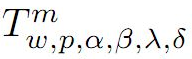in the open unit disc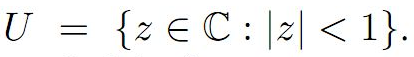We then, introduce a new subclass of analytic function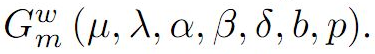Moreover, we discuss coefficient estimates, growth and distortion theorems, and inclusion properties for the functions belonging to the class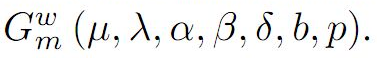2: Paper Source PDF document

Paper's Title:

Algorithms for Nonlinear Problems Involving Strictly Pseudocontractive Mappings

Author(s):

Mathew Olajiire Aibinu1, Surendra Colin Thakur2, Sibusiso Moyo3

1Institute for Systems Science & KZN E-Skill CoLab,
Durban University of Technology,
Durban 4000,
South Africa.

1DSI-NRF Centre of Excellence in Mathematical and Statistical Sciences (CoE-MaSS),
Johannesburg,
South Africa.
E-mail:
moaibinu@yahoo.com mathewa@dut.ac.za

2 KZN E-Skill CoLab,
Durban University of Technology,
Durban 4000,
South Africa.
E-mail:
thakur@dut.ac.za

3Institute for Systems Science & Office of the DVC Research, Innovation & Engagement Milena Court,
Durban University of Technology,
Durban 4000,
South Africa.
E-mail:
dvcrie@dut.ac.za

Abstract:

The puzzles in approximating a fixed point of nonlinear problems involving the class of strictly pseudocontractive mappings are conquered in this paper through viscosity implicit rules. Using generalized contraction mappings, a new viscosity iterative algorithm which is implicit in nature is proposed and analysed in Banach spaces for the class of strictly pseudocontractive mappings. The computations and analysis which are used in the proposed scheme are easy to follow and this gives rooms for a broad application of the scheme. It is obtained that the proposed iterative algorithm converges strongly to a fixed point of a μ-strictly pseudocontractive mapping which also solves a variational inequality problem. The result is also shown to hold for finite family of strictly pseudocontractive mappings. A numerical example is given to show the skillfulness of the proposed scheme and its implementation.

2: Paper Source PDF document

Paper's Title:

Multistage Analytical Approximate Solution of Quasi-Linear Differential- Algebraic System of Index Two

Author(s):

Ibrahim M. Albak, F. A. Abdullah* and Zarita Zainuddin

School of Mathematical Sciences,
Universiti Sains Malaysia,
11800 USM, Penang,
Malaysia.
E-mail:
ibra13975@gmail.com,
farahaini@usm.my,
zarita@usm.my

Abstract:

In this paper, a new Multistage Transform Method (MSDTM) has been proposed by utilizing a well-known transformation technique, the Differential Transform Method (DTM), to solve Differential Algebraic Equations (DAEs) with index 2. The advantage of the proposed scheme is that it does not require an index reduction and extends the convergence domain of the solution. Some examples for various types of problems are carried out to show the ability of MSDTM in solving DAEs. The results obtained are in good agreement with the existing literature which demonstrates the effectiveness and efficiency of the proposed method.

2: Paper Source PDF document

Paper's Title:

Coefficient Bounds for Sakaguchi Kind of Functions Associated with Sine Function

Author(s):

Serap Bulut, H. Priya and B. Srutha Keerth

Kocaeli University,
Faculty of Aviation and Space Sciences,
Arslanbey Campus, 41285 Kartepe-Kocaeli,
Turkey.
E-mail: serap.bulut@kocaeli.edu.tr

Department of Mathematics,
VIT Chennai Campus, Chennai - 600 048,
India.
E-mail: priyaharikrishnan18@gmail.com, priya.h2020@vitstudent.ac.in

Department of Mathematics,
VIT Chennai Campus, Chennai - 600 048,
India.
E-mail: keerthivitmaths@gmail.com, sruthakeerthi.b@vit.ac.in

Abstract:

In this paper, we introduce a new general subclass of analytic functions with respect to symmetric points in the domain of sine function. We obtain sharp coefficient bounds and upper bounds for the Fekete-Szegö functional. Also we get sharp bounds for the logarithmic coefficients of functions belonging to this new class.

2: Paper Source PDF document

Paper's Title:

Higher Order Accurate Compact Schemes for Time Dependent Linear and Nonlinear Convection-Diffusion Equations

Author(s):

S. Thomas, Gopika P.B. and S. K. Nadupuri

Department of Mathematics
National Institute of Technology Calicut
Kerala
673601
India.
E-mail: sobinputhiyaveettil@gmail.com pbgopika@gmail.com nsk@nitc.ac.in

Abstract:

The primary objective of this work is to study higher order compact finite difference schemes for finding the numerical solution of convection-diffusion equations which are widely used in engineering applications. The first part of this work is concerned with a higher order exponential scheme for solving unsteady one dimensional linear convection-diffusion equation. The scheme is set up with a fourth order compact exponential discretization for space and cubic \$C^1\$-spline collocation method for time. The scheme achieves fourth order accuracy in both temporal and spatial variables and is proved to be unconditionally stable. The second part explores the utility of a sixth order compact finite difference scheme in space and Huta's improved sixth order Runge-Kutta scheme in time combined to find the numerical solution of one dimensional nonlinear convection-diffusion equations. Numerical experiments are carried out with Burgers' equation to demonstrate the accuracy of the new scheme which is sixth order in both space and time. Also a sixth order in space predictor-corrector method is proposed. A comparative study is performed of the proposed schemes with existing predictor-corrector method. The investigation of computational order of convergence is presented.

2: Paper Source PDF document

Paper's Title:

Geometrical Properties of Subclass of Analytic Function with Odd Degree

Author(s):

K. Sivagami Sundari and B. Srutha Keerthi

Divison of Mathematics, School of Advanced Sciences,
Vellore Institute of Technology, Chennai Campus, Chennai - 600 127,
India.
E-mail: sivagamisundari.2298@gmail.com

Divison of Mathematics, School of Advanced Sciences,
Vellore Institute of Technology, Chennai Campus, Chennai - 600 127,
India.
E-mail: keerthivitmaths@gmail.com

Abstract:

The objective of the paper is to study the geometrical properties of the class B(λ, t). For which we have proved that the radius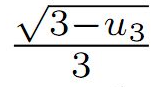is optimal ,(i.e) the numbercannot be replaced by a larger one. Additionally, the graphs for various values of t and λ are compared in order to study the sharpness of the coefficient bounds.

2: Paper Source PDF document

Paper's Title:

Using Direct and Fixed Point Technique of Cubic Functional Equation and its Hyers-Ulam Stability

Author(s):

Ramanuja Rao Kotti, Rajnesh Krishnan Mudaliar, Kaushal Neelam Devi, Shailendra Vikash Narayan

Fiji National University,
Department of Mathematics & Statistics,
P.O. Box 5529, Lautoka,
Fiji.
E-mail: ramanuja.kotti@fnu.ac.fj
URL: https://www.fnu.ac.fj

Abstract:

In this present work, we introduce a new type of finite dimensional cubic functional equation of the form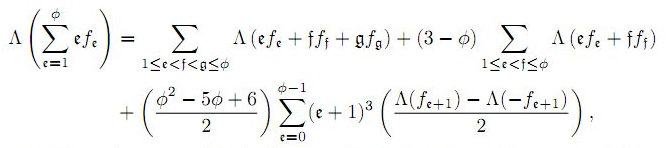where Φ≥4 is an integer, and derive its general solution. The main purpose of this work is to investigate the Hyers-Ulam stability results for the above mentioned functional equation in Fuzzy Banach spaces by means of direct and fixed point methods.

1: Paper Source PDF document

Paper's Title:

New Reverses of Schwarz, Triangle and Bessel Inequalities in Inner Product Spaces

Author(s):

S. S. Dragomir

School of Computer Science and Mathematics, Victoria University of Technology, PO BOX
14428, MCMC 8001, VICTORIA, AUSTRALIA.

sever.dragomir@vu.edu.au
URL
: http://rgmia.vu.edu.au/SSDragomirWeb.html

Abstract:

New reverses of the Schwarz, triangle and Bessel inequalities in inner product spaces are pointed out. These results complement the recent ones obtained by the author in the earlier paper . Further, they are employed to establish new Grüss type inequalities. Finally, some natural integral inequalities are stated as well.

1: Paper Source PDF document

Paper's Title:

On Sufficient Conditions for Strong Starlikeness

Author(s):

V. Ravichandran, M. H. Khan, M. Darus, And K. G. Subramanian

School of Mathematical Sciences, Universiti Sains Malaysia, 11800 Usm Penang, Malaysia
Url
: h
ttp://cs.usm.my/~vravi/index.html

Department of Mathematics, Islamiah College, Vaniambadi 635 751, India
khanhussaff@yahoo.co.in

School of Mathematical Sciences, Faculty of Science and Technology, UKM, Bangi 43600,
M
alaysia
maslina@pkrisc.cc.ukm.my
Url
:

Department of Mathematics, Madras Christian College, Tambaram, Chennai 600 059, India

Abstract:

In the present investigation, we obtain some sufficient conditions for a normalized analytic function f(z) defined on the unit disk to satisfy the condition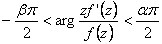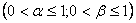1: Paper Source PDF document

Paper's Title:

Fekete-Szegö Inequality for Certain Class of Analytic Functions

Author(s):

V. Ravichandran, Maslina Darus, M. Hussain Khan, and  K. G. Subramanian

School of Mathematical Sciences, Universiti Sains Malaysia,
11800 Usm, Penang, Malaysia

School of Mathematical Sciences, Faculty of Sciences and Technology,
Ukm, Banki 43600, Malaysia

Department of Mathematics, Islamiah College,
V

Department of Mathematics, Madras Christian College, Tambaram,
Chennai- 600 059, India

Abstract:

In this present investigation, the authors obtain Fekete-Szegö inequality for a certain class of analytic functions f(z) for which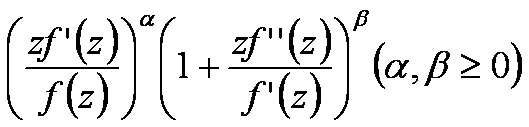lies in a region starlike with respect to 1 and symmetric with respect to the real axis. Also certain application of our main result for a class of functions defined by Hadamard product (convolution) is given. As a special case of our result we obtain Fekete-Szegö inequality for a class of functions defined through fractional derivatives. Also we obtain Fekete-Szegö inequality for the inverse functions.

1: Paper Source PDF document

Paper's Title:

Viability Theory And Differential Lanchester Type Models For Combat.
Differential Systems.

Author(s):

G. Isac and A. Gosselin

Department Of Mathematics, Royal Military College Of Canada,
P.O. Box 17000, S
tn Forces, Kingston, Ontario, Canada K7k 7b4

URL
:

URL
:

Abstract:

In 1914, F.W. Lanchester proposed several mathematical models based on differential equations to describe combat situations . Since then, his work has been extensively modified to represent a variety of competitions including entire wars. Differential Lanchester type models have been studied from many angles by many authors in hundreds of papers and reports. Lanchester type models are used in the planning of optimal strategies, supply and tactics. In this paper, we will show how these models can be studied from a viability theory stand point. We will introduce the notion of winning cone and show that it is a viable cone for these models. In the last part of our paper we will use the viability theory of differential equations to study Lanchester type models from the optimal theory point of view.

1: Paper Source PDF document

Paper's Title:

On Certain Classes of Harmonic Univalent Functions Based on Salagean Operator

Author(s):

G. Murugusundaramoorthy, Thomas Rosy, and B. A. Stephen

Department of Applied Mathematics and Informatics,
Department of Mathematics, Vellore Institute of Technology,
Deemed University, Vellore - 632014, India.
gmsmoorthy@yahoo.com

Department of Applied Mathematics and Informatics,
Department of Mathematics, Madras Christian College,
Chennai - 600059, India.
drthomasrosy@rediffmail.com

Abstract:

We define and investigate a class of complex-valued harmonic univalent functions of the form f = h + g using Salagean operator where h and g are analytic in the unit disc U = { z : |z| < 1 }. A necessary and sufficient coefficient conditions are given for functions in these classes. Furthermore, distortion theorems, inclusion relations, extreme points, convolution conditions and convex combinations for this family of harmonic functions are obtained.

1: Paper Source PDF document

Paper's Title:

A Simple New Proof of Fan-Taussky-Todd Inequalities

Author(s):

Zhi-Hua Zhang and Zhen-Gang Xiao

Zixing Educational Research Section,
Chenzhou City, Hunan 423400, P. R. China.
Zhi-hua Zhang
Url: http://www.hnzxslzx.com/zzhweb/

Department Of Mathematics, Hunan Institute Of Science And Technology,
Yueyang City, Hunan 423400, P. R. China.
Zhen-gang Xiao

Abstract:

In this paper we present simple new proofs of the inequalities: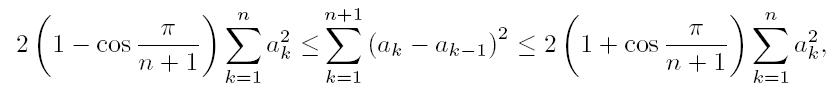which holds for all real numbers a0 = 0, a1, · · · , an, an+1 = 0 and the coefficients 2(1 - cos(π/(n + 1))) and 2(1 + cos(π/(n + 1))) are the best possible; and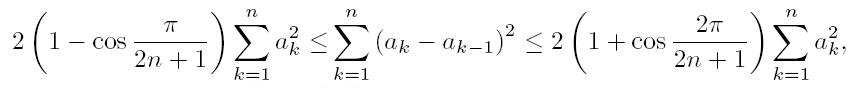which holds for all real numbers a0 = 0, a1, · · · , an and the coefficients 2(1-cos(π/(2n + 1))) and 2(1 + cos(π/(2n + 1))) are the best possible.

1: Paper Source PDF document

Paper's Title:

On a Criteria for Strong Starlikeness

Author(s):

V. Ravichandran, M. Darus, and N. Seenivasagan

School Of Mathematical Sciences, Universiti Sains Malaysia,
11800 Usm Penang, Malaysia
vravi@cs.usm.my
URL: http://cs.usm.my/~vravi

School of Mathematical Sciences, Faculty of Sciences and Technology,
Ukm, Bangi 43600, Malaysia
maslina@pkrisc.cc.ukm.my

Sindhi College, 123, P. H. Road, Numbal,
Chennai 600 077 India
vasagan2000@yahoo.co.in

Abstract:

In this paper, we are concerned with finding sufficient condition for certain normalized analytic function f(z) defined on the open unit disk in the complex plane to be strongly starlike of order α. Also we have obtained similar results for certain functions defined by Ruscheweyh derivatives and Sălăgean derivatives. Further extension of these results are given for certain p-valent analytic functions defined through a linear operator.

1: Paper Source PDF document

Paper's Title:

Refinement Inequalities Among Symmetric Divergence Measures

Author(s):

Inder Jeet Taneja

Departamento de Matemática,
Universidade Federal de Santa Catarina, 88.040-900
Florianópolis, Sc, Brazil

URL: http://www.mtm.ufsc.br/~taneja

Abstract:

There are three classical divergence measures in the literature on information theory and statistics, namely, Jeffryes-Kullback-Leiber’s J-divergence, Sibson-Burbea-Rao’s Jensen- Shannon divegernce and Taneja’s arithemtic - geometric mean divergence. These bear an interesting relationship among each other and are based on logarithmic expressions. The divergence measures like Hellinger discrimination, symmetric χ2divergence, and triangular discrimination are not based on logarithmic expressions. These six divergence measures are symmetric with respect to probability distributions. In this paper some interesting inequalities among these symmetric divergence measures are studied. Refinements of these inequalities are also given. Some inequalities due to Dragomir et al.  are also improved.

1: Paper Source PDF document

Paper's Title:

Boundedness for Vector-Valued Multilinear Singular Integral Operators on Triebel-Lizorkin Spaces

Author(s):

Liu Lanzhe

College of Mathematics
Changsha University of Science and Technology,
Changsha 410077,
P.R. of China.
lanzheliu@263.net

Abstract:

In this paper, the boundedness for some vector-valued multilinear operators associated to certain fractional singular integral operators on Triebel-Lizorkin space are obtained. The operators include Calderón-Zygmund singular integral operator and fractional integral operator.

1: Paper Source PDF document

Paper's Title:

On Zeros of Diagonally Quasiconvex Multifunctions

Author(s):

Zoran D. Mitrović

Faculty of Electrical Engineering,
University of Banja Luka,
78000 Banja Luka, Patre 5
Bosnia and Herzegovina
zmitrovic@etfbl.net

Abstract:

In this paper, we extended the notion of diagonally quasiconvexity for multifunctions and established several existence results for zeros of diagonally quasiconvex multifunctions. As applications we obtain the results of fixed points, coincidence points and best approximations for multifunctions. Using our result we also prove the existence of solutions to the variational-like inequality problem and generalized vector equilibrium problem. The results of this paper generalize some known results in the literature.

1: Paper Source PDF document

Paper's Title:

Iterated Order of Fast Growth Solutions of Linear Differential Equations

Author(s):

Benharrat Belaďdi

Department of Mathematics
Laboratory of Pure and Applied Mathematics
University of Mostaganem
B. P. 227 Mostaganem,
ALGERIA.
belaidi@univ-mosta.dz

Abstract:

In this paper, we investigate the growth of solutions of the differential equation f(k) + Ak-1 (z) f(k-1) +...+ A1 (z) f' + A0 (z) f= F (z), where Ao (z), ..., Ak-1 (z) and F (z)0 are entire functions. Some estimates are given for the iterated order of solutions of the above quation when one of the coefficients As is being dominant in the sense that it has larger growth than Aj (j≠s) and F.

1: Paper Source PDF document

Paper's Title:

A Coincidence Theorem for Two Kakutani Maps

Author(s):

Mircea Balaj

Department of Mathematics,
Romania.

Abstract:

In this paper we prove the following theorem: Let X be a nonempty compact convex set in a locally convex Hausdorff topological vector space, D be the set of its extremal points and F,T: XX two Kakutani maps; if for each nonempty finite subset A of D and for any x ∈ coA, F (x) coA ≠ Ř, then F and T have a coincidence point. The proof of this theorem is given first in the case when X is a simplex, then when X is a polytope and finally in the general case. Several reformulations of this result are given in the last part of the paper.

1: Paper Source PDF document

Paper's Title:

Topological Aspects of Scalarization in Vector Optimization Problems.

Author(s):

Peter I. Kogut, Rosanna Manzo and Igor V. Nechay

Department of Differential Equations,
Dnipropetrovsk National University, Naukova STR.,
13, 49010 Dnipropetrovsk,
Ukraine

p.kogut@i.ua

Universitŕ di Salerno,
Dipartimento di Ingegneria dell'Informazione e Matematica Applicata,
Via Ponte don Melillo, 84084 Fisciano (SA),
Italy

manzo@diima.unisa.it

Department of Technical Cybernetics,
Dnipropetrovsk Technical University,
Acad. Lazarjan STR., 2, 49010 Dnipropetrovsk,
Ukraine

i.nechay@i.ua

Abstract:

In this paper, we study vector optimization problems in partially ordered Banach spaces. We suppose that the objective mapping possesses a weakened property of lower semicontinuity and make no assumptions on the interior of the ordering cone. We derive sufficient conditions for existence of efficient solutions of the above problems and discuss the role of topological properties of the objective space. We discuss the scalarization of vector optimization problems when the objective functions are vector-valued mappings with a weakened property of lower semicontinuity. We also prove the existence of the so-called generalized efficient solutions via the scalarization process. All principal notions and assertions are illustrated by numerous examples.

1: Paper Source PDF document

Paper's Title:

On an Elliptic Over-Determined Problem in Dimension Two

Author(s):

Lakhdar Ragoub
Department of Mathematics and Information of Tiyadhechnology
AL Yamamah University
P.O. Box 45 180, Riyadh 11 512
Saudi Arabia.

lragoub@yu.edu.sa

Abstract:

We extend the method of Weinberger for a non-linear over-determined elliptic problem
in R2. We prove that the domain in consideration is a ball. The tool of this investigation are maximum principles and P-functions.

1: Paper Source PDF document

Paper's Title:

Existence Results for Second Order Impulsive Functional Differential Equations with Infinite Delay

Author(s):

M. Lakrib, A. Oumansour and K. Yadi

Laboratoire de Mathématiques, Université Djillali
Liabées, B.P. 89 Sidi Bel Abbčs 22000, Algérie
mlakrib@univ-sba.dz
oumansour@univ-sba.dz

Laboratoire de Mathématiques, Université Abou Bekr
Belkaid, B.P. 119 Tlemcen 13000, Algérie

Abstract:

In this paper we study the existence of solutions for second order impulsive functional differential equations with infinite delay. To obtain our results, we apply fixed point methods.

1: Paper Source PDF document

Paper's Title:

Some Distortion and Other Properties Associated with a Family of the n-Fold Symmetric Koebe Type Functions

Author(s):

H. M. Srivastava, N. Tuneski and E. Georgieva-Celakoska

Department of Mathematics and Statistics, University of Victoria,
Victoria, British Columbia V8W 3R4,

harimsri@math.uvic.ca

Faculty of Mechanical Engineering, St.
Cyril and Methodius University,
Karpo'v s II b.b., MK-1000 Skopje,
Republic of Macedonia

Abstract:

In a recent work by Kamali and Srivastava , a certain family of the n-fold symmetric Koebe type functions was introduced and studied systematically. In an earlier investigation, Eguchi and Owa  had considered its special case when n=1 (see also ). Here, in our present sequel to these earlier works, this general family of the n-fold symmetric Koebe type functions is studied further and several distortion theorems and such other properties as the radii of spirallikeness, the radii of starlikeness and the radii of convexity, which are associated with this family of the n-fold symmetric Koebe type functions, are obtained. We also provide certain criteria that embed this family of the n-fold symmetric Koebe type functions in a function class Gλ which was introduced and studied earlier by Silverman .

1: Paper Source PDF document

Paper's Title:

Properties of Certain Multivalent Functions Involving Ruscheweyh Derivatives

Author(s):

N-Eng Xu and Ding-Gong Yang

Department of Mathematics,
Changshu Institute of Technology,
Changshu, Jiangsu 215500,
China

Abstract:

Let Ap(p∈ N) be the class of functions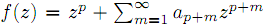which are analytic in the unit disk. By virtue of the Ruscheweyh derivatives we introduce the new subclasses Cp(n,α,β,λ,μ) of Ap. Subordination relations, inclusion relations, convolution properties and a sharp coefficient estimate are obtained. We also give a sufficient condition for a function to be in Cp(n,α,β,λ,μ)

1: Paper Source PDF document

Paper's Title:

Some Properties of the Marshall-Olkin and Generalized Cuadras-Augé Families of Copulas

Author(s):

Edward Dobrowolski and Pranesh Kumar

Department of Mathematics and Statistics
University of Northern British Columbia
Prince George, BC,

E-mail: Pranesh.Kumar@unbc.ca

Abstract:

We investigate some properties of the families of two parameter Marshall-Olkin and Generalized Cuadras-Augé copulas. Some new results are proved for copula parameters, dependence measure and mutual information. A numerical application is discussed.

1: Paper Source PDF document

Paper's Title:

On the Sendov Conjecture for a Root Close to the Unit Circle

Author(s):

Indraneel G. Kasmalkar

Department of Mathematics,
University of California,
Berkeley, CA 94720
United States of America

E-mail: indraneelk@berkeley.edu

Abstract:

On Sendov's conjecture, T. Chijiwa quantifies the idea stated by V. Vâjâitu and A. Zaharescu (and M. J. Miller independently), namely that if a polynomial with all roots inside the closed unit disk has a root sufficiently close to the unit circle then there is a critical point at a distance of at most one from that root. Chijiwa provides an estimate of exponential order for the required 'closeness' of the root to the unit circle so that such a critical point may exist. In this paper, we will improve this estimate to polynomial order by making major modifications and strengthening inequalities in Chijiwa's proof.

1: Paper Source PDF document

Paper's Title:

Hermite-Hadamard-Fejer Type Inequalities for Harmonically s-convex Functions via Fractional Integrals

Author(s):

İmdat İşcan, Mehmet Kunt

Department of Mathematics,
Faculty of Sciences and Arts,
Giresun University, Giresun,
Turkey.
E-mail: imdat.iscan@giresun.edu.tr

Department of Mathematics,
Faculty of Sciences,
61080, Trabzon,
Turkey.
E-mail: mkunt@ktu.edu.tr

Abstract:

In this paper, some Hermite-Hadamard-Fejer type integral inequalities for harmonically s-convex functions in fractional integral forms have been obtained.

1: Paper Source PDF document

Paper's Title:

A Note on Divergent Fourier Series and λ-Permutations

Author(s):

A. Castillo, J. Chavez and H. Kim

Tufts University,
Department of Mathematics,
Medford, MA 02155,
USA
E-mail: angel.castillo@tufts.edu

Texas Tech University,
Department of Mathematics and Statistics,
Lubbock, TX 79409,
USA
E-mail: josechavez5@my.unt.edu

University of Michigan-Dearborn,
Department of Mathematics and Statistics,
Dearborn, MI 48128,
USA.
E-mail: khyejin@umich.edu

Abstract:

We present a continuous function on [-π,π] whose Fourier series diverges and it cannot be rearranged to converge by a λ-permutation.

1: Paper Source PDF document

Paper's Title:

New Refinements of Hölder's Inequality

Author(s):

Xiu-Fen Ma

College of Mathematical and Computer,
No.9 of Xuefu Road, Hechuan District 401520,
Chongqing City,
The People's Republic of China.
E-mail: maxiufen86@163.com

Abstract:

In this paper, we define two mappings, investigate their properties, obtain some new refinements of Hölder's inequality.

1: Paper Source PDF document

Paper's Title:

On Singular Numbers of Hankel Matrices of Markov Functions

Author(s):

Vasily A. Prokhorov

Department of Mathematics and Statistics,
University of South Alabama,
Mobile, Alabama 36688-0002,
USA.
E-mail: prokhoro@southalabama.edu
URL: http://www.southalabama.edu/mathstat/people/prokhorov.shtml

Abstract:

Let E ⊂ (01,1) be a compact set and let μ be a positive Borel measure with support supp μ=E. Let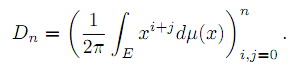In the case when E=[a,b]⊂ (-1,1) and μ satisfies the condition dμ/dx>0 a.e. on E, we investigate asymptotic behavior of singular numbers σkn,n of the Hankel matrix Dn, where kn/n→θ∈[0,1] as n→∞. Moreover, we obtain asymptotics of the Kolmogorov, Gelfand and linear k-widths, k=kn, of the unit ball An,2 of Pn∩L2(Γ) in the space L2(μ,E), where Γ={z:|z|=1} and Pn is the class of all polynomials of the degree at most n.

1: Paper Source PDF document

Paper's Title:

Wavelet Frames in Higher Dimensional Sobolev Spaces

Author(s):

Raj Kumar, Manish Chauhan, and Reena

Department of Mathematics,
Kirori Mal College, University of Delhi,
New Delhi-110007,
India.
E-mail: rajkmc@gmail.com

Department of Mathematics,
University of Delhi,
New Delhi-110007,
India
E-mail: manish17102021@gmail.com

Department of Mathematics,
Hans Raj College, University of Delhi,
New Delhi-110007,
India
E-mail: reena.bhagwat29@gmail.com

Abstract:

In this paper, we present sufficient condition for the sequence of vectors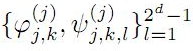to be a frame for Hs(Rd) are derived. Necessary and sufficient conditions for the sequence of vectorsto be tight wavelet frames in Hs(Rd) are obtained. Further, as an application an example of tight wavelet frames for Hs(R2) as bivariate box spline over 3-direction are given.

1: Paper Source PDF document

Paper's Title:

MSplit Equality for Monotone Inclusion Problem and Fixed Point Problem in Real Banach Spaces

Author(s):

1,2Christian Chibueze Okeke, 3Abdumalik Usman Bello, 1Chinedu Izuchukwu, and 1Oluwatosin Temitope Mewomo

1School of Mathematics,
Statistics and Computer Science,
University of KwaZulu-Natal, Durban,
South Africa.
E-mail: okekec@ukzn.ac.za
E-mail: izuchukwuc@ukzn.ac.za
E-mail: mewomoo@ukzn.ac.za

2DST-NRF Center of Excellence in Mathematical and Statistical Sciences (CoE-Mass)
Johannesburg,
South Africa.

3Federal University,
Dutsin-Ma, Katsina State,
Nigeria.
E-mail: uabdulmalik@fudutsinma.edu.ng

Abstract:

In this paper a new iterative algorithm for approximating a common solution of split equality monotone inclusion problem and split equality fixed point problem is introduced. Using our algorithm, we state and prove a strong convergence theorem for approximating an element in the intersection of the set of solutions of a split equality monotone inclusion problem and the set of solutions of a split equality fixed point problem for right Bregman strongly nonexpansive mappings in the setting of p-uniformly convex Banach spaces which are also uniformly smooth. We also give some applications.

1: Paper Source PDF document

Paper's Title:

Polynomial Dichotomy of C0-Quasi Semigroups in Banach Spaces

Author(s):

Sutrima1,2, Christiana Rini Indrati2, Lina Aryati2

1Department of Mathematics,
Universitas Sebelas Maret,
PO Box 57126, Surakarta,
Indonesia.
E-mail: sutrima@mipa.uns.ac.id

2Department of Mathematics,
PO Box 55281, Yogyakarta,
Indonesia.
E-mail: rinii@ugm.ac.id, lina@ugm.ac.id

Abstract:

Stability of solutions of the problems is an important aspect for application purposes. Since its introduction by Datko , the concept of exponential stability has been developed in various types of stability by various approaches. The existing conditions use evolution operator, evolution semigroup, and quasi semigroup approach for the non-autonomous problems and a semigroup approach for the autonomous cases. However, the polynomial stability based on C0-quasi semigroups has not been discussed in the references. In this paper we propose a new stability for C0-quasi semigroups on Banach spaces i.e the polynomial stability and polynomial dichotomy. As the results, the sufficient and necessary conditions for the polynomial and uniform polynomial stability are established as well as the sufficiency for the polynomial dichotomy. The results are also confirmed by the examples.

1: Paper Source PDF document

Paper's Title:

The Influence of Fluid Pressure in Macromechanical Cochlear Model

Author(s):

F. E. Aboulkhouatem1, F. Kouilily1, N. Achtaich1, N. Yousfi1 and M. El Khasmi2

1Department of Mathematics and Computer Science, Faculty of Sciences
Ben M'sik, Hassan II University, Casablanca,
Morocco.

2Department of Biology, Faculty of Sciences
Ben M'sik, Hassan II University, Casablanca,
Morocco.

E-mail: fatiaboulkhouatem@gemail.com
URL: http://www.fsb.univh2c.ma/

Abstract:

An increase of pressure in the structure of cochlea may cause a hearing loss. In this paper, we established the relationship between the fluid pressure and the amplitude of displacement of Basilar Membrane to clarify the mechanisms of hearing loss caused by increasing of this pressure. So, a mathematical cochlear model was formulated using finite difference method in order to explain and demonstrate this malfunction in passive model. Numerical simulations may be considered as helpful tools which may extend and complete the understanding of a cochlea dysfunction.

1: Paper Source PDF document

Paper's Title:

A Generalization of Ostrowski's Inequality for Functions of Bounded Variation via a Parameter

Author(s):

Seth Kermausuor

Department of Mathematics and Computer Science,
Alabama State University,
Montgomery, AL 36101,
USA.
E-mail: skermausour@alasu.edu

Abstract:

In this paper, we provide a generalization of the Ostrowski's inequality for functions of bounded variation for k points via a parameter λ∈[0,1]. As a by product, we consider some particular cases to obtained some interesting inequalities in these directions. Our results generalizes some of the results by Dragomir in [S. S. DRAGOMIR, The Ostrowski inequality for mappings of bounded variation, Bull. Austral. Math. Soc., 60 (1999), pp. 495--508.]

1: Paper Source PDF document

Paper's Title:

Some New Mappings Related to Weighted Mean Inequalities

Author(s):

Xiu-Fen Ma

College of Mathematical and Computer,
No.9 of Xuefu Road, Hechuan District 401520,
Chongqing City,
The People's Republic of China.
E-mail: maxiufen86@163.com

Abstract:

In this paper, we define four mappings related to weighted mean inequalities, investigate their properties, and obtain some new refinements of weighted mean inequalities.

1: Paper Source PDF document

Paper's Title:

Generalised Models for Torsional Spine Reconnection

Author(s):

Ali Khalaf Hussain Al-Hachami

Department of Mathematics,
College of Education For Pure Sciences,
Wasit University,
Iraq.
E-mail: alhachamia@uowasit.edu.iq

Abstract:

Three-dimensional (3D) null points are available in wealth in the solar corona, and the equivalent is probably going to be valid in other astrophysical situations. On-going outcomes from sun oriented perceptions and from reproductions propose that reconnection at such 3D nulls may assume a significant job in the coronal dynamics. The properties of the torsional spine method of magnetic reconnection at 3D nulls are researched. Kinematic model are created, which incorporate the term ηJ that is spatially localised around the null, stretching out along the spine of the null. The null point is to research the impact of shifting the level of asymmetry of the null point magnetic field on the subsequent reconnection process where past examinations constantly considered a non-nonexclusive radially symmetric null. Specifically we analyse the rate of reconnection of magnetic flux at the spine of null point. Logical arrangements are determined for the enduring kinematic equation, and contrasted and the after effects of torsional spine reconnection models when the current is restricted in which the Maxwell conditions are illuminated. The geometry of the current layers inside which torsional spine reconnection happen is autonomous on the symmetry of the magnetic field. Torsional spine reconnection happens in a thin cylinder around the spine, with circular cross-segment when the fan eigenvalues are extraordinary. The short axis of the circle being along the solid field bearing. Just as it was discovered that the fundamental structure of the method of attractive reconnection considered is unaffected by changing the magnetic field symmetry, that is, the plasma flow is discovered rotational around the spine of null point. The spatiotemporal pinnacle current, and the pinnacle reconnection rate achieved, are found not to rely upon the level of asymmetry.

1: Paper Source PDF document

Paper's Title:

Author(s):

F. U. Ogbuisi

School of Mathematics, Statistics and Computer Science,
University of KwaZulu-Natal, Durban,
South Africa.

Department of Mathematics,
University of Nigeria, Nsukka,
Nigeria.
E-mail: ferdinard.ogbuisi@unn.edu.ng fudochukwu@yahoo.com

Abstract:

In this paper, we propose and analyze a type of subgradient extragradient algorithm for the approximation of a solution of variational inequality problem which is also a common fixed point of an infinite family of relatively nonexpansive mappings in 2-uniformly convex Banach spaces which are uniformly smooth. By using the generalized projection operator, we prove a strong convergence theorem which does not require the prior knowledge of the Lipschitz constant of cost operator. We further applied our result to constrained convex minimization problem, convex feasibility problem and infinite family of equilibrium problems. Our results improve and complement related results in 2-uniformly convex and uniformly smooth Banach spaces and Hilbert spaces.

1: Paper Source PDF document

Paper's Title:

Optimal Control Analysis of HIV/AIDS Epidemic Model with an Antiretroviral Treatment

Author(s):

U. Habibah and R. A. Sari

Mathematics Department and Reseach Group of Biomathematics,
Faculty of Mathematics and Natural Science,
Brawijaya University, Jl. Veteran Malang 65145,
Indonesia.
E-mail: ummu_habibah@ub.ac.id

Abstract:

A mathematical model of HIV/AIDS is governed by a system of ordinary differential equations in the presence of an antiretroviral treatment (ARV). The theory of optimal control is applied to an epidemic model of HIV/AIDS which an ARV is used as a control strategy in order to prevent the spread of HIV/AIDS. The optimality system is derived by applying the Pontryagin's Minimum Principle. We analyze the boundedness and positivity of solutions, and an existence of the optimal control. Numerical simulations are conducted to obtain numerical solution of the optimally system.

1: Paper Source PDF document

Paper's Title:

Estimates of Norms on Krein Spaces

Author(s):

Satheesh K. Athira, P. Sam Johnson and K. Kamaraj

Department of Mathematical and Computational Sciences,
National Institute of Technology Karnataka,
Surathkal, Mangaluru 575 025,
India.
E-mail: athirachandri@gmail.com

Department of Mathematical and Computational Sciences,
National Institute of Technology Karnataka,
Surathkal, Mangaluru 575 025,
India.
E-mail:sam@nitk.edu.in

Department of Mathematics,
University College of Engineering Arni,
Anna University, Arni 632 326,
India.
E-mail: krajkj@yahoo.com

Abstract:

Various norms can be defined on a Krein space by choosing different underlying fundamental decompositions. Some estimates of norms on Krein spaces are discussed and a few results in Bognar's paper are generalized.

1: Paper Source PDF document

Paper's Title:

Existence and Approximation of Traveling Wavefronts for the Diffusive Mackey-Glass Equation

Author(s):

C. Ramirez-Carrasco and J. Molina-Garay

Chile
E-mail: carloshrc1989@gmail.com
molina@imca.edu.pe

Abstract:

In this paper, we consider the diffusive Mackey-Glass model with discrete delay. This equation describes the dynamics of the blood cell production. We investigate the existence of traveling wavefronts solutions connecting the two steady states of the model. We develop an alternative proof of the existence of such solutions and we also demonstrate the existence of traveling wavefronts moving at minimum speed. The proposed approach is based on the use technique of upper-lower solutions. Finally, through an iterative procedure, we show numerical simulations that approximate the traveling wavefronts, thus confirming our theoretical results.

1: Paper Source PDF document

Paper's Title:

A Note on Schur's Lemma in Banach Function Spaces

Author(s):

R. E. Castillo, H. Rafeiro and E. M. Rojas

Departamento de Matematicas, Bogota,
Colombia.
E-mail: recastillo@unal.edu.co

United Arab Emirates University,
Department of Mathematical Sciences, Al Ain,
United Arab Emirates.
E-mail: rafeiro@uaeu.ac.ae

Departamento de Matematicas, Bogota,
Colombia.
E-mail: emrojass@unal.edu.co

Abstract:

In this small note, in a self contained presentation, we show the validity of Schur's type lemma in the framework of Banach function spaces.

1: Paper Source PDF document

Paper's Title:

Locally Bicomplex Convex Module and Their Applications

Author(s):

Department of Mathematics,
University of Jammu,
Jammu And Kashmir,
India.
E-mail: stanzinkunga19@gmail.com

Department of Mathematics,
University of Jammu,
Jammu And Kashmir,
India.

Abstract:

Let X be a locally BC convex module and L(X) be the family of all continuous bicomplex linear operators on X. In this paper, we study some concepts of D-valued seminorms on locally BC convex module. Further, we study the bicomplex version of Co and (Co,1) semigroup. The work of this paper is inspired by the work in  and .

1: Paper Source PDF document

Paper's Title:

Trace Inequalities for Operators in Hilbert Spaces: a Survey of Recent Results

Author(s):

Sever S. Dragomir1,2

1Mathematics, School of Engineering & Science
Victoria University,
PO Box 14428 Melbourne City, MC 8001,
Australia
E-mail: sever.dragomir@vu.edu.au

2DST-NRF Centre of Excellence in the Mathematical and Statistical Sciences,
School of Computer Science & Applied Mathematics,
University of the Witwatersrand,
Private Bag 3, Johannesburg 2050,
South Africa
URL: https://rgmia.org/dragomir

Abstract:

In this paper we survey some recent trace inequalities for operators in Hilbert spaces that are connected to Schwarz's, Buzano's and Kato's inequalities and the reverses of Schwarz inequality known in the literature as Cassels' inequality and Shisha-Mond's inequality. Applications for some functionals that are naturally associated to some of these inequalities and for functions of operators defined by power series are given. Further, various trace inequalities for convex functions are presented including refinements of Jensen inequality and several reverses of Jensen's inequality. Hermite-Hadamard type inequalities and the trace version of Slater's inequality are given. Some Lipschitz type inequalities are also surveyed. Examples for fundamental functions such as the power, logarithmic, resolvent and exponential functions are provided as well.

1: Paper Source PDF document

Paper's Title:

Corrigendum for Multistage Analytical Approximate Solution of Quasi-Linear Differential- Algebraic System of Index Two

Author(s):

Ibrahim M. Albak, F. A. Abdullah* and Zarita Zainuddin

School of Mathematical Sciences,
Universiti Sains Malaysia,
11800 USM, Penang,
Malaysia.
E-mail:
ibra13975@gmail.com,
farahaini@usm.my,
zarita@usm.my

Abstract:

1: Paper Source PDF document

Paper's Title:

Preserver of Local Spectrum of Skew-product Operators

Author(s):

1Department of Mathematics,
College of Sciences,
University of Yasouj,
Yasouj, 75918-74934,
Iran.
E-mail: r.parvinian@yu.ac.ir

2Department of Mathematics,
College of Sciences,
University of Yasouj,
Yasouj, 75918-74934,
Iran.

3Department of Mathematics,
Ghor Institute of higher education,
Afghanistan.
E-mail: jumapazhman@gmail.com

Abstract:

Let H and K be infinite-dimensional complex Hilbert spaces, and B(H) (resp. B(K)) be the algebra of all bounded linear operators on H (resp. on K). For an operator T B(H) and a vector h H, let σT(h) denote the local spectrum of T at h. For two nonzero vectors h0 H and k0 K, we show that if two maps φ1 and φ2 from B(H) into B(K) satisfy

σφ1(T)φ2(S)*(k0)= σTS*(h0})

for all T, S B(H), and their range containing all operators of rank at most two, then there exist bijective linear maps P : H K and Q : K H such that φ1(T) = PTQ and φ2(T)* =Q-1T*P-1 for all T B(H). Also, we obtain some interesting results in this direction.

1: Paper Source PDF document

Paper's Title:

Application of Chebyshev Polynomials to Volterra-Fredholm Integral Equations

Author(s):

Department of Mathematics,
Faculty of Mathematics and
Informatics,
University of Msila,
Algeria.

E-mail: aissa.lakhal@univ-msila.dz

Abstract:

The goal of this work is to examine the numerical solution of linear Volterra-Fredholm integral equations of the second kind using the first, second, third and fourth Chebyshev polynomials. Noting that, the approximate solution is given in the form of series which converges to the exact one. Numerical examples are compared with other methods, in order to prove the applicability and the efficiency of this technical.

1: Paper Source PDF document

Paper's Title:

Bicomplex Univalent Functions

Author(s):

Mohd Arif, Amjad Ali, Rajat Singh* and Romesh Kumar

Department of Mathematics,
University of Jammu,
Jammu And Kashmir,
India.
E-mail: azizymaths@gmail.com

Department of Mathematics,
University of Jammu,
Jammu And Kashmir,
India.

Department of Mathematics,
University of Jammu,
Jammu And Kashmir,
India.
E-mail: *rajat.singh.rs634@gmail.com

Department of Mathematics,
University of Jammu,
Jammu And Kashmir,
India.
E-mail: romeshmath@gmail.com

Abstract:

In this paper we introduce bicomplex univalent functions and also discuss the properties of a specific class of univalent functions.

1: Paper Source PDF document

Paper's Title:

A Self Adaptive Method for Solving Split Bilevel Variational Inequalities Problem in Hilbert Spaces

Author(s):

Francis Akutsah1, Ojen Kumar Narain2, Funmilayo Abibat Kasali3 Olawale Kazeem Oyewole4 and Akindele Adebayo Mebawondu5

1School of Mathematics,
Statistics and Computer Science,
University of KwaZulu-Natal, Durban,
South Africa.
E-mail: 216040405@stu.ukzn.ac.za, akutsah@gmail.com

2School of Mathematics,
Statistics and Computer Science,
University of KwaZulu-Natal, Durban,
South Africa.
E-mail: naraino@ukzn.ac.za

3Mountain Top University,
Prayer City, Ogun State,
Nigeria.
E-mail: fkasali@mtu.edu.ng

4Technion-Israel Institute of Technology.
E-mail: 217079141@stu.ukzn.ac.za, oyewoleolawalekazeem@gmail.co

5School of Mathematics,
Statistics and Computer Science,
University of KwaZulu-Natal, Durban,
South Africa.
DST-NRF Centre of Excellence in Mathematical and Statistical Sciences (CoE-MaSS),
Johannesburg,
South Africa.
Mountain Top University,
Prayer City, Ogun State,
Nigeria.
E-mail: dele@aims.ac.za

Abstract:

In this work, we study the split bilevel variational inequality problem in two real Hilbert spaces. We propose a new modified inertial projection and contraction method for solving the aforementioned problem when one of the operators is pseudomonotone and Lipschitz continuous while the other operator is α-strongly monotone. The use of the weakly sequential continuity condition on the Pseudomonotone operator is removed in this work. A Strong convergence theorem of the proposed method is proved under some mild conditions. In addition, some numerical experiments are presented to show the efficiency and implementation of our method in comparison with other methods in the literature in the framework of infinite dimensional Hilbert spaces. The results obtained in this paper extend, generalize and improve several.

Search and serve lasted 1 second(s).